## Table of ContentOpen Access

ARTICLE

# Towards a Unified Single Analysis Framework Embedded with Multiple Spatial and Time Discretized Methods for Linear Structural Dynamics

David Tae, Kumar K. Tamma*

Department of Mechanical Engineering, University of Minnesota, Twin Cities, Minneapolis, 55455, USA

* Corresponding Author: Kumar K. Tamma. Email:Computer Modeling in Engineering & Sciences 2023, 135(2), 843-885. https://doi.org/10.32604/cmes.2023.023071

## Abstract

We propose a novel computational framework that is capable of employing different time integration algorithms and different space discretized methods such as the Finite Element Method, particle methods, and other spatial methods on a single body sub-divided into multiple subdomains. This is in conjunction with implementing the well known Generalized Single Step Single Solve (GS4) family of algorithms which encompass the entire scope of Linear Multistep algorithms that have been developed over the past 50 years or so and are second order accurate into the Differential Algebraic Equation framework. In the current state of technology, the coupling of altogether different time integration algorithms has been limited to the same family of algorithms such as the Newmark methods and the coupling of different algorithms usually has resulted in reduced accuracy in one or more variables including the Lagrange multiplier. However, the robustness and versatility of the GS4 with its ability to accurately account for the numerical shifts in various time schemes it encompasses, overcomes such barriers and allows a wide variety of arbitrary implicit-implicit, implicit-explicit, and explicit-explicit pairing of the various time schemes while maintaining the second order accuracy in time for not only all primary variables such as displacement, velocity and acceleration but also the Lagrange multipliers used for coupling the subdomains. By selecting an appropriate spatial method and time scheme on the area with localized phenomena contrary to utilizing a single process on the entire body, the proposed work has the potential to better capture the physics of a given simulation. The method is validated by solving 2D problems for the linear second order systems with various combination of spatial methods and time schemes with great flexibility. The accuracy and efficacy of the present work have not yet been seen in the current field, and it has shown significant promise in its capabilities and effectiveness for general linear dynamics through numerical examples.

## Keywords

1  Introduction

As numerical analysis is utilized in the various fields and applications, the desire for analyzing complex problems more efficiently has grown. The Finite Element Method (FEM) is one of the most widely used numerical analysis methods. While it features simplicity and versatility, it is susceptible to numerical errors such as shear locking and complexity in adopting it for large deformation problems and the like.

Concurrently, the strong form based particle methods has been gaining interest in the field of numerical simulations as the particle methods have the capability and flexibility for handling large distortion, crack propagation and free surface detecting, etc. Some of the notable strong form particle methods, Smoothed Particle Hydrodynamics (SPH)  and Moving Particle Semi-implicit Method (MPS) [5,6], have been used to solve partial differential equations. Later, Silling et al. proposed a nonlocal extension of classical continuum mechanics called peridynamics (PD) [7,8], which represents spatial derivatives of field quantities in terms of internal interactions between points/particles via integrals involving differences. The peridynamics model shows a comprehensive ability to solve linear and nonlinear second order system problems and simulate structural dynamics and molecular dynamics as well as multi-scale problems . More recently, a mixed form representation of the strong form particle method, where multiple primary variables are solved at once, is presented in . Other related works appear in references .

As problems become more complex, controlling the spatial discretization locally is a desirable feature. While an area where fast dynamics are presented is discretized with high spatial resolutions, a coarser mesh may be sufficient in the majority of the structure. In addition, the selection of spatial methods in different parts of a body can also be an effective strategy such as by utilizing the FEM for the majority of a body, and applying particle based methods only on an area where localized phenomena such as crack or fracture may occur.

One of the ways to locally control spatial discretization is dividing a body into regions of subdomains in which different methods can be applied in each subdomain. The challenge in using subdomains is handling the interface condition between the subdomains, and over the years, numerous coupling methods have been developed. Interface coupling is largely divided into two categories: overlapping and non-overlapping domain coupling. In the case of the overlapping domain decomposition, a portion of the subdomains are overlapped and the shape function similar to the framework of the FEM is used to constrain the common Degrees of Freedom (DOFs). However, the large difference in each of the subdomain’s stiffness may lead to the global stiffness matrix to be ill-conditioned, as noted by Orsini et al. in . Moreover, Metsis et al.  implied that the weak form based domain decomposition methods may be more prone to this issue due to the implementation of the Gaussian integral.

On the other hand, the non-overlapping technique is more straight forward where the subdomains are only connected by an edge or a surface in 2D or 3D, respectably, and are constrained by the Lagrange multiplier at the interface. At the interface, usually the nodes/particles are overlapped; however, Park et al.  and Brezzi et al.  introduced an approach where the subdomains are not directly interacting but through an intermediate interface. This approach allows more flexibility in meshing as one-to-one matching of the nodes is not required.

Implementing the different spatial discretization methods for localized phenomena has been a study which has been explored for many years. The coupling of the FEM with molecular dynamics via a bridging domain which enforces compatibility by utilizing the Lagrange multiplier is proposed by Xiao et al. . Alternately, the Discrete Element Method (DEM)  is a widely used mesh-free method in the rock engineering simulation field and various FEM-DEM coupling methods have been developed [21,22]. Utilizing the peridynamics method for localized phenomena such as crack propagation is also a topic with extensive studies including coupling the FEM and the PD . In this work, one to one node/particle matching is used to simply demonstrate the concept of coupling different spatial methods and different time integration schemes unlike state of art which is limited to just the spatial methods. We couple the FEM and particle methods with different time schemes for each subdomain and such features are not feasible in the state of art.

There also has been high interest in coupling subdomains for transient analysis. Gravouil et al. presented the GC method in , employing different time increments in subdomains using the limited Newmark family of algorithms. However, it was later discovered that artificial numerical dissipation arises at the interface resulting in the loss of energy and loss of second order accuracy in the GC method.

Similarly, Pegon extended the GC method and proposed the PM method which employs an inter-field parallel solution procedure to couple different subdomains [27,28]. Prakash et al.  developed the PH method in order to alleviate the GC method’s energy issue by solving the interface at a large time scale. The drawback of the PH method was described by Karimi et al. who stated in , that the PH method can only apply to two subdomains. Karimi et al.  developed the subdomain DAE framework for the Newmark family of algorithms. A noteworthy aspect of this work is that the constrained continuous system in time is in fact a system of differential algebraic equations rather than ordinary differential equations in time which most coupling methods have been based upon. Nakshatrala et al. later applied the DAE approach to the Finite Element Tearing and Interconneting (FETI) method based domain decomposition to solve time dependent first order systems with the fourth order Nakshatrala et al. . More recently, Shimada  integrated the Generalized Single Step Single Solve (GS4) family of algorithms with the subdomain DAE framework using the FEM. And, Maxam et al.   proposed an unified approach of the DAE-GS4 framework on thermoelastic problems using the FEM. There exists interest in coupling different subdomains for transient analysis. However, the current algorithm technology is very limited and does not permit features to interface a wide variety of time algorithms and spatial methods. And, this is the focus of the advances and contributions of this paper.

In this work, the subdomain DAE framework is particularly advanced to the Generalized Single Step Single Solve (GSSSS or GS4) framework and family of algorithms developed by Tamma et al. . The versatility of the GSSSS family of algorithms in the treatment of numerical dissipation, overshoot and ease of adapting to explicit methods, proves extremely useful to the subdomain framework. In addition, the coupling of the FEM based on the weak form, and the generalized particle method (GPS) based on the strong form is also presented in this work to demonstrate integrating altogether different spatial methods as well. The coupling of different spatial and time discretization methods may lead to more accurate computation as it allows the selection of the appropriate method for a specific situation. The novel GS4 framework and its family of algorithms is a general purpose structure. It encompasses the entire class of Linear Multistep (LMS) methods including those that have been developed over the past 50 years or so and in addition, also includes new and advanced optimal designs of algorithms, in 1st/2nd order systems that are second order time accurate and used for commercial software to enable practical real word applications to be conducted. In addition, under one umbrella, the frameworks inherit and have new and optimal algorithms and designs in addition to existing methods such as Crank-Nicolson, Gear’s BDF, etc. for first order systems; and new and optimal choices in second order systems as well in contrast to existing ones such as the Newmark family, HHT, WBZ and the like. A particularly unique feature is that the framework permits interfacing a wide variety of implicit-implicit, implicit-explicit, and explicit-explicit algorithm designs in a single analysis unlike the existing methods which are limited and not capable of doing so; in particular coupling of different implicit-implicit algorithms with/without controllable numerical dissipation which is not feasible in the current state-of-the-art. This is a significant breakthrough and is capitalized herein. The generality, flexibility and robustness of the present computational framework which embraces subdomain and time integration algorithms and differing spatial methods fused into one is not possible with today’s traditional methods and technology that shows limitations such as reduced order accuracy, consistency problems and the like in various field variables such as displacement, velocity, acceleration and Lagrange multiplier. The present developments provide a wide variety of choices to the analyst. In this work, we consistently preserve and maintain second order time accuracy in all variables such as displacement, velocity and acceleration including the Lagrange multipliers. In this paper, the FEM and GPS are first reviewed to explain the basics in integrating different spatial methods as well as different time integration methods in a single analysis. Then in the following section, the incorporation of the GS4 family of algorithms with the DAE framework is investigated and validated through various numerical examples.

2  Finite Element Method

A brief overview of the finite element method is reviewed in this section for better understanding of the various numerical examples and its methodology that are presented in a later section. In addition, the objective is to get clarity of the computations involved in the calculations of the FEM integrals and particle method integrals.

2.1 Finite Element Approximations

In the finite element method, a body of domain Ω is divided into numerous regions called elements formed by connecting points known as nodes. The finite dimensional field variable such as the displacement uh is approximated within each element by the basis functions. The most common basis functions have the Kronecker delta δij property so that the nodal values u^ can be accurately represented. The integration of the finite dimension is performed by summing up the integrals over each element domain.

Ωg(u)dΩ=j=1NhΩjg(uh)dΩj=j=1NelemΩjg(Niu^i)dΩj(1)

where Ni are the elemental basis functions which varies for different types of element. The basis functions for a commonly used 2D bilinear quadrilateral are given as follows:

u=Niu^i=[N1(ξ,η)N2(ξ,η)N3(ξ,η)N4(ξ,η)][u1u2u3u4]T(2)

where

N1(ξ,η)=14(1ξ)(1η)N2(ξ,η)=14(1+ξ)(1η)N3(ξ,η)=14(1+ξ)(1+η)N4(ξ,η)=14(1ξ)(1+η)(3)

Quadrilateral elements with two Gauss points in each x and y direction will be used throughout in the numerical example section.

2.2 Weak Form Formulation for FEM

The equilibrium equation expressed in terms of the displacement can be written as (shown for 1D as a simple illustration)

σx+bx=ρ2ut2(4)

Next we introduce an arbitrary function w(x) that is defined in the domain Ω and multiply the equilibrium equation.

g(w,u,σx)=w(x)(ρ2ut2σxbx)=0(5)

Then we integrate over the domain to obtain an integral form that is set to zero.

G(w,u,σx)=Ωw(x)(ρ2ut2σxbx)dx=0(6)

The stress term is then integrated by parts as

Ωw(x)σxxdx=Ωwxσxdxw(x)nxσx|Γ(7)

where Γ is the boundary of Ω and nx is the outward pointing normal to the boundary. By substitution of Eq. (7) into Eq. (6), we get the weak form of the equilibrium as

G(w,u,σx)=Ωw(x)(ρ2ut2bx)dx+Ωwxσxdxwtx|Γuwt¯x|Γt=0(8)

Introduce the constitutive equation to obtain governing equation based on displacement, and we get

G(w,u)=Ωw(x)(ρ2ut2bx)dx+ΩwxEuxdxwt¯x|Γt=0(9)

It is worth noting that we removed tx by setting w = 0 where the displacements are specified.

The resulting weak formulation in space is given as

ρΩw2ut2dΩ+ΩwEudΩ=ΩwbdΩ+ΩΓuwt¯dΓ(10)

and limiting the trial and test spaces to high fidelity finite element spaces, we get

ρΩwh2uht2dΩ+ΩwhEuhdΩ=ΩwhbdΩ+ΩΓuwht¯dΓ(11)

Setting uh=Niu^hi and wh=Niw^hi, for the Ritz-Galerkin approximation, we have

ρw^hiTΩNTNu^¨hidΩ+w^hiTΩNTENu^hidΩ=w^hiTΩNTbdΩ+w^hiTΓNTt¯dΓ(12)

The above equation is valid for any w^h. Therefore we may write

ρΩNTNu^¨hidΩ+EΩNTNu^hidΩ=ΩNTbdΩ+ΓNTt¯dΓ(13)

Mu^¨hi+Ku^hi=F^(14)

M=ρΩNTNdΩ(15)

K=EΩNTNdΩ(16)

F^=ΩNTbdΩ+ΓNTt¯dΓ(17)

3  Generalized Particle Method

3.1 Gradient and Divergence of Classical Physical Field

A physical field can be thought of as the assignment of a physical quantity at each point in space and time. Consider a domain in Euclidean space, E. Let XΩ0E denotes the geometric position vector of a certain position in the domain and X_Ω0E denotes another point which is close to the position X as shown in Fig. 1a. Therefore, any physical quantity at each point in space and time can be defined as φ(X,t):Ω0×IE. Define ΩXΩ0 as a domain where the center point position vector X is in, then we have

φ(X,t)=limVΩX0VΩXφ(X_,t)dVVΩX(18)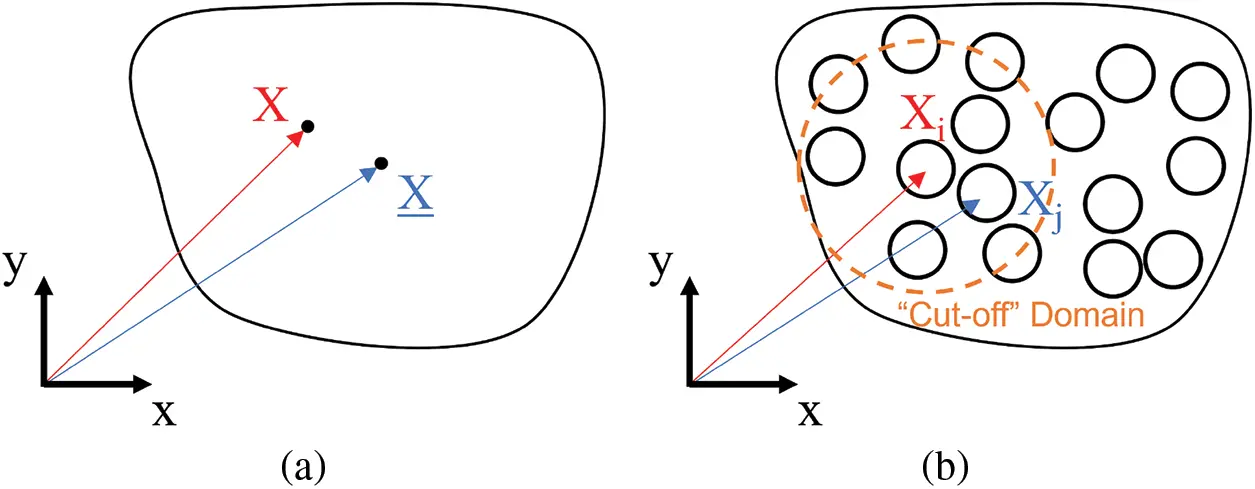Figure 1: (a) Continuous domain with position vector X and X, (b) Discretized domain with position vectors Xi and Xj

The weighted residual method has been widely used in solving different kinds of ordinary/partial differential equations, in particular, using the Galerkin method, which is the fundamental theory of the finite element method. In this work, we exploit the weighted residual method directly to the Taylor series expansion, such that a generalized approach of developing particle based methods can be explored.

In mathematics, the Taylor series expansion is a representation of a function as an infinite sum of terms that are calculated from the values of the function’s derivatives at a single point. The generalized Taylor series of a general physical quantity φt(X_) about X at a fixed time t yields

φ(X_,t)=φ(X,t)+Λ1φ(X,t)(X_X)+Λ2H(X,t)(X_X)(X_X)++O(X_Xn)(19)

where φ(X,t) is a vector when φ(X,t) is a scalar value and is a second order tensor when φ(X,t) is a vector value; and ΛiR(i=1,2,3.) is the algorithmic parameters.

Omitting the higher order terms from Eq. (19), we have the first order Taylor series approximation

φ(X_,t)φ(X,t)Λ1φ(X,t)(X_X)(20)

where Λ1=1. Define a residual with regards to Eq. (20), R, as

R=φ(X_,t)φ(X,t)φ(X,t)(X_X)(21)

According to the standard weighted residual method, we introduce a weight function, C1, and minimize the residual. Eq. (21) is multiplied by the weight function C and integrated over the domain ΩX, which then yields

ΩXRCdV=0(22)

It is worth noting that the domain is the cut-off influence domain of the position X, and the residual is a local value. The weight function C provides a wide scope of approximations for constructing the derivative definitions used in various particle-based methods and new approach of particle methods as well.

Gradient Recall the residual R in Eq. (21) and the weighted residual, RC, with respect to a vector property φ is

(RC)XX_=(φ(X_,t)φ(X,t))Cφ(X,t)XX_(X_X)C(23)

where C is a vector with the size of geometric dimension of a problem; for example, C is a two by one vector in 2D problems. We postulate that Eq. (23) is the exact relationship between the point X and one of its neighboring point X_ located in the domain ΩX in terms of the weighted residual, (RC)XX_. Therefore, by integrating Eq. (23) over the domain ΩX, the following formulations can be obtained as

VΩXRCdV=VΩX[(φ(X_,t)φ(X,t))C]φ(X,t)XX_[(X_X)C]dV=0(24)

By treating (φ(X_,t)φ(X,t))C and φ(X,t)(X_X)C in the integral presented above as field properties in the domain ΩX, the following formulations can be obtained as

1VΩXVΩX[(φ(X_,t)φ(X,t))C]dV=1VΩXVΩXφ(X,t)XX_[(X_X)C]dV(25)

Hence, the gradient of a vector can be obtained as

φ(X,t)=limVΩX0(VΩX(φ(X_,t)φ(X,t))CdV)limVΩX0(VΩX(X_X)CdV)1(26)

The proposed gradient operator has the ability to recover the in the Moving Particle Semi-implicit (MPS) method, the deformation gradient in the state-based peridynamics as well as in the corrected Smoothed Particle Hydrodynamics (SPH) method, by selecting the appropriate corresponding C.

Remark 3.1. By selecting the arbitrary C=nij, the Gradient in terms of the original MPS method can be derived from the proposed approach as well. Substituting C=nij in the discretized system yields,

φi=[jΩXi(XjXi)nijVj]1[jΩXi(φjφi)nijVj](27)

Let A=(X_X)nij and it can be replaced by its normalized weighted average value within an infinitesimal domain via exploiting Eq. (18),

AXX_avg=1VΩXlimVΩ0ΩX[(X_X)C]dV(28)

Discretizing into particles, and applying nij=(XjXi)/||XjXi||, it becomes

Aij=1VΩXijΩXi(nijnij)Vj(||XjXi||)=jΩXi(nijnij)WijVjVΩXijΩXiWijNormalizedWeightedAverageA(||XjXi||)(29)

Thus, Eq. (27) can be written as

φij=[kΩXj(njknjk)WjkVkVΩXjkΩXjWjk]1(φjφi)nij||XjXi||(30)

Introducing the weighting function Wij and supposing Vi=Vj,VΩXi=VΩXj yields

φi=1VΩXijΩXiWijjΩXi[kΩXj(njknjk)WjkVkVΩXjkΩXjWjk]Ajk1(φjφi)nij||XjXi||WijVj=1jΩXiWijjΩXi[kΩXj(njknjk)WjkkΩXjWjk]1(φjφi)nij||XjXi||Wij(31)

It is worth noting that for a general particle within a uniformly distributed system, the weighted average matrix Ajk is a diagonal matrix. Consequently, Ajk=dI, where I is identity matrix and d equals to the dimensional number. Therefore, Eq. (31) recovers the gradient calculation in the MPS method, and can be rewritten as

φi=dn0jΩXi(φjφi)||XjXi||2(XjXi)Wij(32)

where n0=jWij.

Divergence By representing the divergence model as gradient operator, ∇, dot product with a physical quantity, φ, the divergence model can be written as following

φ(X,t)=Tφ=limVΩX0(VΩX(φ(X_,t)φ(X,t))CdV)limVΩX0(VΩX(X_X)CdV)1(33)

The discretized formulations is listed as following

φi=[jΩXi(φjφi)CjWijVj][jΩXi(XjXi)CjWijVj]1(34)

Laplacian The definition of the Laplacian can be written as

2φ(X,t)=φ(X,t)(35)

In order to obtain the Laplacian relationship between the center point X and its neighboring point X_, we reconstruct the gradient between two points via taking advantage of Eq. (26). One may notice that it is convenient to find the gradient from Eq. (23) by setting the (RC)XX_ as 0, which yields

φ(X,t)XX_=[(φ(X_,t)φ(X,t))C][(X_X)C]1(36)

However, the rank of matrix [(X_X)C] is 1, which is not available for the calculation of the inverse when the gradient is carried over from the vector gradient calculation. To circumvent the issue, we introduce A=(X_X)C. Then it can be replaced by its average value within an infinitesimal domain via exploiting Eq. (18),

AXX_avg=1VΩXlimVΩX0ΩX[(X_X)C]dV(37)

Therefore, an alternative gradient of a vector is given by taking advantage of the average property, Eq. (37).

φ(X,t)XX_=[(φ(X_,t)φ(X,t))C](AXX_avg)1(38)

Consequently, the Laplacian can be obtained via combining Gauss’s Theorem and the gradient formulations.

(φ(X,t))XX_VX_=φ(X_,t)nXX_SX_(39)

where S_=V_||X_X||. As will be explained in the later section, we can also replace nXX_ with C. Substitution of S_ into Eq. (39) yields

(φ(X,t))XX_||X_X||=φ(X_,t)C(40)

Therefore, the Laplacian of φ(X,t) becomes

(φ(X,t))XX_||X_X||=[(φ(X_,t)φ(X,t))C](AXX_avg)1C(41)

Hence the following Laplacian formulations can be obtained as

2φ(X,t)=φ(X,t)=limVΩX01VΩXΩX[(φ(X_,t)φ(X,t))C]AXX_avg1C||X_X||dV(42)

The discretized formulations is listed as following:

2φi=1VΩXijΩXi[(φjφi)Cj]Aijavg1Cj||XjXi||WijVj(43)

3.2 Stiffness Matrix for Particle Method

We will explore how the stiffness matrix is formed in 2D using the GPS method. We start with the same governing equation and constitutive law,

σ=Fσ=Du(44)

where D is elastic matrix as following

D=E1ν2[1ν0ν10001ν2]for plane stress(45)

D=E(1+ν)(12ν)[1νν0ν1ν00012ν]for plane strain(46)

The first step to get the stiffness matrix is to describe ∇u with the gradient operator from the GPS method as described in Eq. (32).

ui=[jΩXi(ujui)CijWijVj][jΩXi(XjXi)CijWijVj]1[A](47)

where [A] is a two by two matrix that is computed first. The summation is applied for every particle within particle i’s influence circle. But for simplicity, we will consider there are two particles for the system, particles 1 and 2. The Eq. (47) will be written as following for particle 1,

u1=[(u2xu1x)(u2yu1y)][(C12x)(C12y)][A11A12A21A22]W12V2(48)

=[C12x(u2xu1x)C12y(u2xu1x)C12x(u2yu1y)C12y(u2yu1y)][A11A12A21A22]W12V2(49)

Thus,

[u12xxu12xyu12yxu12yy]=[(A11C12x+A21C12y)(u2xu1x)(A12C12x+A22C12y)(u2xu1x)(A11C12x+A21C12y)(u2yu1y)(A12C12x+A22C12y)(u2yu1y)]W12V2(50)

Next, we need to convert the 2 by 2 matrix on the left hand side of Eq. (50) to a 4 by 1 vector form.

[u12xxu12xyu12yxu12yy]=W12V2[(A11C12x+A21C12y)(u2xu1x)(A12C12x+A22C12y)(u2xu1x)(A11C12x+A21C12y)(u2yu1y)(A12C12x+A22C12y)(u2yu1y)]=W12V2[(A11C12x+A21C12y)0(A11C12x+A21C12y)0(A12C12x+A22C12y)0(A12C12x+A22C12y)00(A11C12x+A21C12y)0(A11C12x+A21C12y)0(A12C12x+A22C12y)0(A12C12x+A22C12y)]×[u1xu1yu2xu2y](51)

We can also get the engineering strain as follows:

Eq. (52) can be simply written as

[ϵ1]=[u](53)

Stress σ can be computed by multiplying elastic matrix, [D], from Eqs. (45) and (46).

[σ1]=[D][u](54)

It is worth noting that [D][∇][u] includes all the components in the particle i’s influence domain.

[σ]=[D][][u]=[[D][D][D][n]][u](55)

where  and  represent the gradient operator obtained from the first and the second particle as the i th particle, respectively. This suggests that, in general, the gradient operator for every particle in the system needs to be computed before applying divergence operator.

Next, we will show how to construct the divergence operator into a matrix form similar to how the gradient operator matrix was formed as shown above.

σ1=[C12xC12y][A11A12A21A22][σ112σ111σ122σ121σ212σ211σ222σ221]W12V2(56)

=[A11C12x+A21C12yA12C12x+A22C12y][σ112σ111σ122σ121σ212σ211σ222σ221]W12V2(57)

=[B1(σ112σ111)B2(σ122σ121)B1(σ212σ211)B2(σ222σ221)](58)

where B1=(A11C12x+A21C12y) and B2=(A12C12x+A22C12y) for simplicity. Here we further isolate σ together.

[σ1xσ1y]=W12V2[B1B200B1B20000B1B200B1B2][σ111σ121σ211σ221σ112σ122σ212σ222](59)

or for the engineering stress,

[σ1xσ1y]=W12V2[B10B2B10B20B2B10B2B1][]:divergence operator for particle 1[σxx1σyy1τxy1σxx2σyy2τxy2](60)

Thus, we can get the stiffness matrix for the particle 1, by combining Eqs. (55) and (60),

[][D][][u]=FW12V2[B10B2B10B20B2B10B2B1][[D][D]][K1][[u]]=[F1xF1y](61)

This process is repeated for each particle in the system. After generating [Ki] matrices, they are assembled together to form the system stiffness matrix [K].

4  Equations of Motion for a System of Multiple Subdomains

Differential Algebraic Equations (DAE) are differential equations with algebraic constraints. Consider the equation of motion of a body that is subject to constraints g(u) = 0. The action integral is given as

S(u,λ)=t0tfLt+λTg(u)(62)

and we can take the variation of the action integral,

δS(u,λ)=0t(L(u,u.)u.)L(u,u.)u+GT(u)λ=0(63)

g(u)=0(64)

where G(u) = ∂g(u)/u. For the standard DAE equations of second order transient systems, the above equation can be written as

Qiner(u,u.,u..)+Qint(u)=Qappl(u,u.,t)+GT(u)λ(65)

g(u)=0(66)

where, Qiner, Qint and Qappl are the inertial, internal and applied forces, respectively. The Lagrange multiplier variable, λ(t), is introduced in order to accomplish the constraint equation.

Now, consider a domain of interest Ω that is divided into ndom number of subdomains, Ωi (for i=1,2,,ndom), with each subdomain joined by a non-overlapping interface in between. An example with three subdomains is shown in Fig. 2. In the case of linear semidiscrete second order transient systems, the equation of motion for subdomain Ωi is, (for i=1,2,,ndom),

Miu..i=Qiint(ui)+Qiappl(ui,u.i,t)+GiTλ(t)(67a)

0=i=1ndomGiu.i(67b)

where ui(t), u.i(t):=dui/dt, and u..i(t):=d2ui/dt2 are the displacement, velocity, and acceleration vectors of the ith-subdomain, respectively; Mi is the mass matrix of the ith-subdomain; and Qiint(ui) and Qiappl(ui,u.i,t) are the internal and applied force of the ith-subdomain, respectively.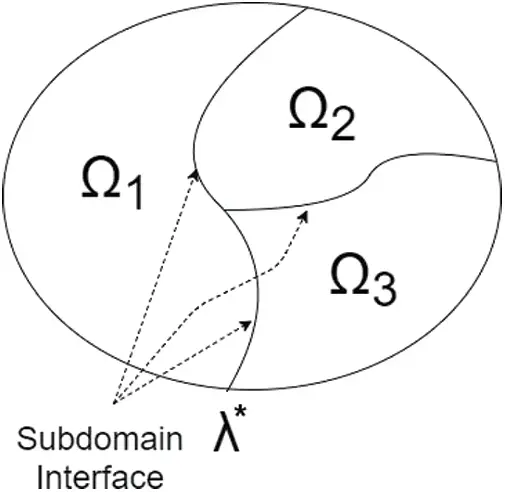Figure 2: A domain Ω divided into three subdomains with non-overlapping interface

The matrix Gi is a boolean matrix with entries either −1, 0, or +1, that is defined by the subdomain interface conditions. The nodes or particles on one side of the interface will have −1 while the corresponding nodes or particles on the other side of the interface will have value of +1 and all other nodes/particles will have 0. Eq. (67b) indicates that the velocity continuity along the interface is enforced.

4.1 DAE Framework with GS4 Family of Algorithms for a System with Multiple Subdomains and Multiple Time Step Sizes

Let Δt and Δti be the system time step size and the subdomain time step size for Ωi, respectively, and define the ratio as τi=Δt/Δti1 for i=1,2,,ndom. The system and subdomain time step sizes are defined as

Δt=tn+1tn(68a)

Δti=tn+m+1τitn+mτi(68b)

where n denotes the system time step, and m represents subdomain time step. The visual representation of time subcylcing is shown in Fig. 3.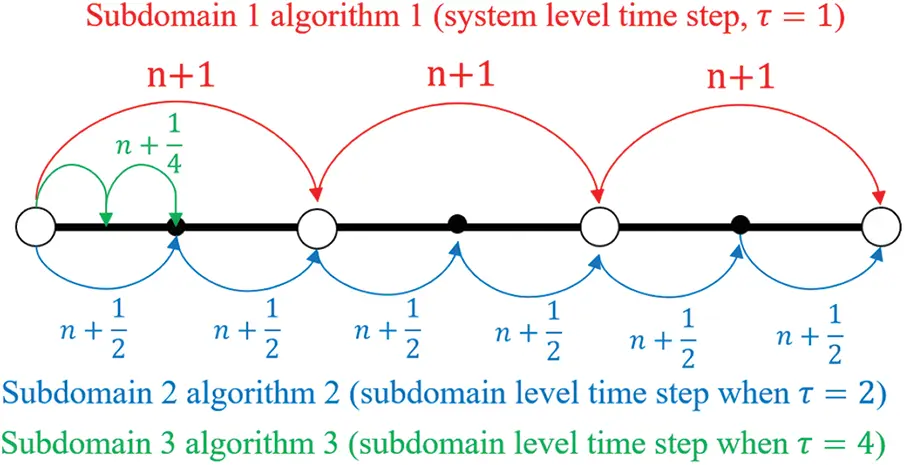Figure 3: Time step subcycling for different subdomains

Now applying the GS4 family of algorithms, the equation of motion of each subdomain can be written as following:

Mia~i(n,m)=Qiint(u~i(n,m))+Qiappl(u~i(n,m),v~i(n,m),tn+m+W1τi)+GiTλ~(n,m)(69a)

0=i=1ndomGivin+1(69b)

where

tn+m+W1τi:=tn+(m+W1τi)Δt=t0+nΔt+(m+W1)Δti(69c)

λ~(n,m):=λn+(m+W1τi)(λn+1λn)(69d)

a~i(n,m):=ain+mτi+W1Λ6Δai(n,m)(69e)

v~i(n,m):=vin+mτi+ΔtW1Λ4ain+mτi+ΔtW2Λ5Δai(n,m)(69f)

u~i(n,m):=uin+mτi+ΔtW1Λ1vin+mτi+Δt2W2Λ2ain+mτi+Δt2W3Λ3Δai(n,m)(69g)

It is worth noting that the Lagrange multipliers are approximated in the system time level rather than in the subdomain time level. This factor suggests that the solution must be in the system time level even though the displacement, velocity, and acceleration are approximated in the subdomain time step.

The updates of each of the unknown variables with the GS4 framework of algorithms are

uin+m+1τi=uin+mτi+Δtλ1vin+mτi+Δt2λ2ain+mτi+Δt2λ3Δai(n,m)(70a)

vin+m+1τi=vin+mτi+Δtλ4ain+mτi+Δtλ5Δai(n,m)(70b)

ain+m+1τi=ain+mτi+Δai(n,m)(70c)

WΛ and λ are the GS4 algorithmic parameters and can be found in . The advantage of the GS4 algorithm is that it allows one to easily switch between various time integration algorithms as well as explicit and implicit families based on the GS4 algorithmic parameters. A single analysis code readily enables current and future researchers and analysts to investigate a multitude of algorithms and combinations in the academic and commercial software world; thereby making it a much desired computational simulation toolkit.

In linear dynamical systems, Eq. (67) may be written as

Mia~i+Civ~i+Kiu~i=Qiappl(t)+GiTλ~(t)(71a)

0=i=1ndomGiu.in+1(71b)

where Ci and Ki are the damping and stiffness matrices for Ωi, respectively; and Qiappl(t) is the external force vector for Ωi. Since the stiffness matrix is different for each subdomain, various methods can be coupled together with each subdomain having the stiffness matrix derived from the corresponding methods. For example, subdomain 1 and 2 in Fig. 2 can use the stiffness matrix derived from the FEM while subdomain 3 adopts the stiffness matrix formulated using the GPS method as outlined in the previous section.

A set of all kinematic unknowns for Ωi in its subdomain time step is defined as

Xin+mτi:=[ain+mτivin+mτiuin+mτi](72)

Eq. (71a) can then be discretized and written as follows:

LiXin+m+1τi(m+W1τi)(G^i(1))T(λn+1)=Qin+m+W1τi+(1m+W1τi)(G^i(1))Tλn+RiXin+mτi(73)

where

Li:=[LiOOΔtiλ5IIOΔti2λ3IOI]withLi:=W1Λ6Mi+ΔtiW2Λ5Ci+Δti2W3Λ3Ki(74)

Ri:=[Ri(1+ΔtiW1Λ1)IIΔti(λ4λ5)IIOΔti2(λ2λ3)IΔtiλ1II](75a)

with

Ri:=(W1Λ61)Mi+Δti(W2Λ5W1Λ4)Ci+Δti2(W3Λ3W2Λ2)Ki(75b)

Qin+m+W1τi:=[Qi(tn+m+W1τi)00](76)

and

G^i(1):=[GiOnc×niOnc×ni](77)

where O represent zero-matrix, nc and ni refer to the number of constraint degrees of freedom, and total number of degrees of freedom in one domain, respectively. Now, we define a set of all kinematic unknowns, i.e., the acceleration, velocity, and displacement vectors, for Ωi over its subdomain time step and a set of all kinematic unknowns of all subdomains in the system over a system time step as

Xi(n+1):=[Xin+1τiXin+2τiXin+τi1τiXin+1]andX(n+1):=[X1(n+1)X2(n+1)Xndom(n+1)](78)

The constraint equation at the velocity level, i.e., Eq. (71b), can be written in the form

0=i=1ndomGiXi(n+1)=GX(n+1)(79)

where

G:=[G1G2Gndom](80)

and

Gi:=[1τiOnc×3ni2τiOnc×3niτi1τiOnc×3niG^i](81)

with

G^i:=[Onc×niGiOnc×ni](82)

Eq. (82) shows the velocity constraint. The size of Gi is nc by 3niτi since the system solves for the displacement, velocity and acceleration at every subcycling step simultaneously. However, Eq. (81) suggests that the constraint is enforced only at the n+1 time step.

The equation of motion for the entire system for a system time step level can be represented in a simple matrix form as following:

[ABGO][X(n+1)λn+1]=[F(n)0](83)

where

A:=diag[A1,A2,,Andom](84)

in which

Ai:=[LiRiLiRiLiRiLi](85)

B:=[B1B2Bndom](86)

in which

Bi:=[1τiG^i(1)2τiG^i(1)τi1τiG^i(1)G^i(1)]T(87)

and

F(n):=[F1(n)F2(n)Fndom(n)](88)

in which

Fi(n):=[Qin+1+W1τi+(1G^i(1))Tλn+RiXinQin+2+W1τi+(1G^i(1))TλnQin+τi+W1τi+(1G^i(1))Tλn](89)

4.2 Computational Procedure

The computation flowchart for the GS4-II DAE framework for the subdomain method employing different spatial methods, algorithms, and time steps is shown below in Fig. 4 which here which provides a wide variety of algorithms and choices as options to the analyst.

for dom := 1 to Ndom do

Calculate M, C, K using corresponding method for the domain

Calculate Li, and Ri using Eqs. (74) and (75a)

Calculate Ĝi using Eq. (82)

for τ := 1 to n do

Assemble Ai using Eq. (85)

Assemble Bi using Eq. (87)

end for

end for

Assemble A using Eq. (84)

Assemble B using Eq. (86)

Transpose B to obtain G

for (t := 1 to Timesteps) do

for dom := 1 to Ndom do

Calculate Fi using Eq. (89)

end for

Assemble F using Eq. (88)

Assemble ^Rglob and Ĵglob from Eq. (83)

Solve (ĴglobXn+1 = ^Rglob)

end for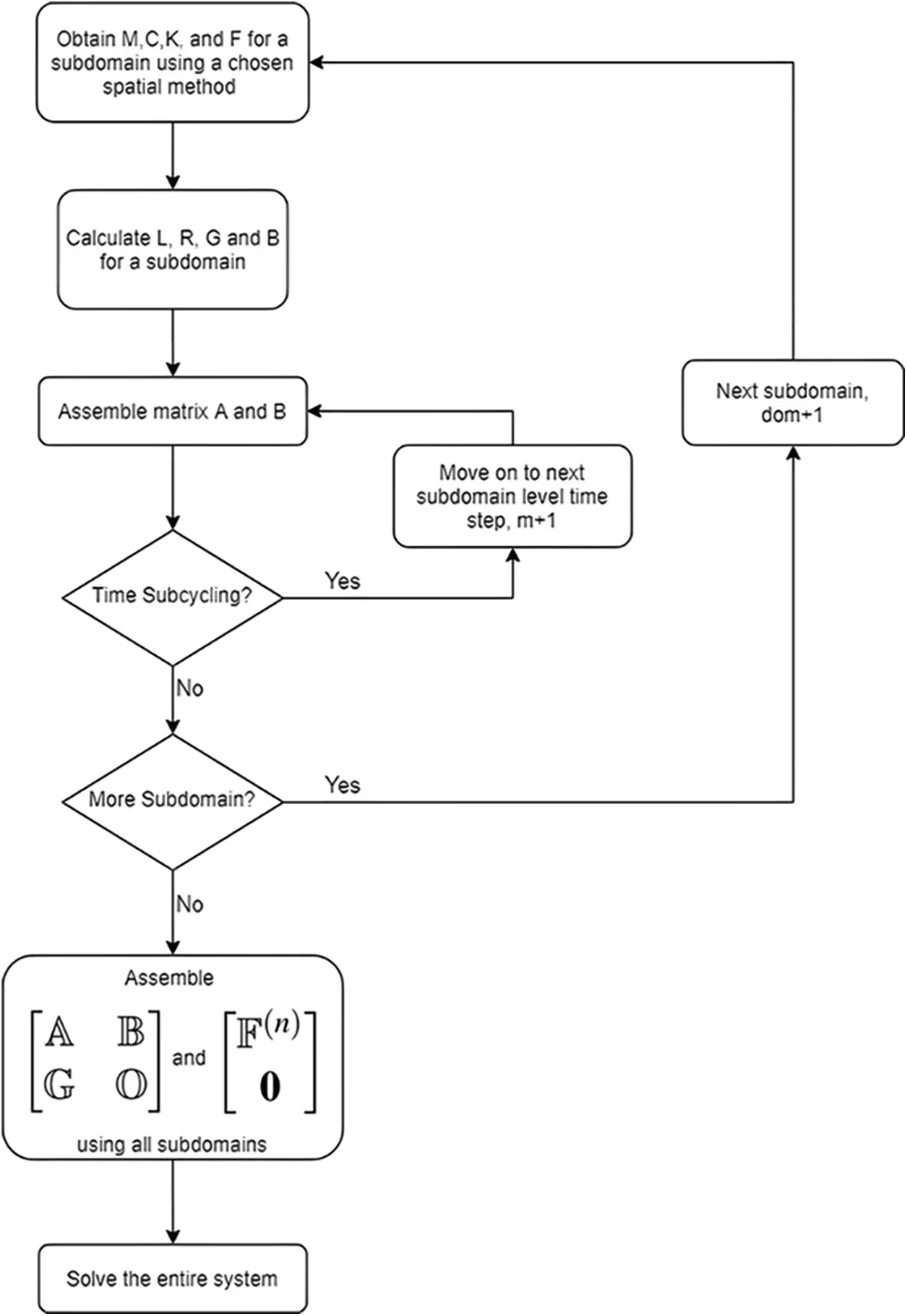Figure 4: Flowchart for constructing DAE second order system

4.3 Subdomain Coupling Condition and Time Level Consistency for Second Order Systems

The second order time accuracy of all the primary variables and the Lagrange multipliers can be achieved for interfacing different subdomains (this is in general not plausible with traditional methods) if W1, a parameter in GS4, is the same for all subdomains. In order to guarantee the second order time accuracy in the acceleration, the algorithmic acceleration ain+mτi must be an approximation at time level tn:

ain+mτiu..(tn+(mϕi)Δti)(90)

where ϕi:=W1Λ6W1 in which W1 and W1Λ6 are the values used for subdomain Ωi.

The GS4-II algorithms are written in the form of U0(ρmin,ρmax,ρs) for the U0 family and V0 (ρmin,ρmax,ρs) for the V0 family. The relation of the variables follows 0ρsρminρmax1. And depending on the value given to each variable, it recovers various existing time schemes including a wide variety of new and optimal methods and also provides new avenues to integrate multiple methods in a single analysis; for example, U0(1, 1, 0) recovers Newmark time scheme, and U0(0, 0, 0) is equal to the WBZ scheme. The V0 family represents an altogether new family of algorithms and is not well known to others and is relatively new and not well understood by the traditional research community at large; mathematically, the V0 family of algorithms are the mirror counterparts of the U0 family but with different overshoot behavior unlike several of the traditional methods. More recent efforts show the advances in the GS4 V0* family of algorithms that enable consistent second order time accurate features with and without physical damping . Certain algorithms within the U0 family are also new with certain exceptions which are existing methods. The GS4 encompasses the entire family of LMS methods that are second order time accurate. Only three parameters are needed to identify a particular algorithm; and no two algorithms can have the same DNA (Discrete Numerically Assigned markers).

With the W1 parameter condition, the GS4-II family of time integration scheme combinations guarantee second order time accuracy of all variables and the Lagrange multipliers; this is unlike existing technology which does not permit such implicit interfacing of arbitrary methods whilst consistently preserving second order accuracy. Some of the possible GS4-II time scheme combinations are listed in Table 1. For example, one cannot simply or haphazardly couple the Midpoint rule with the Newmark method; or couple the Newmark, HHT, WBZ methods, etc., arbitrarily in different subdomains in a single analysis without loss and reduced accuracy in the convergence rate of one or more variables.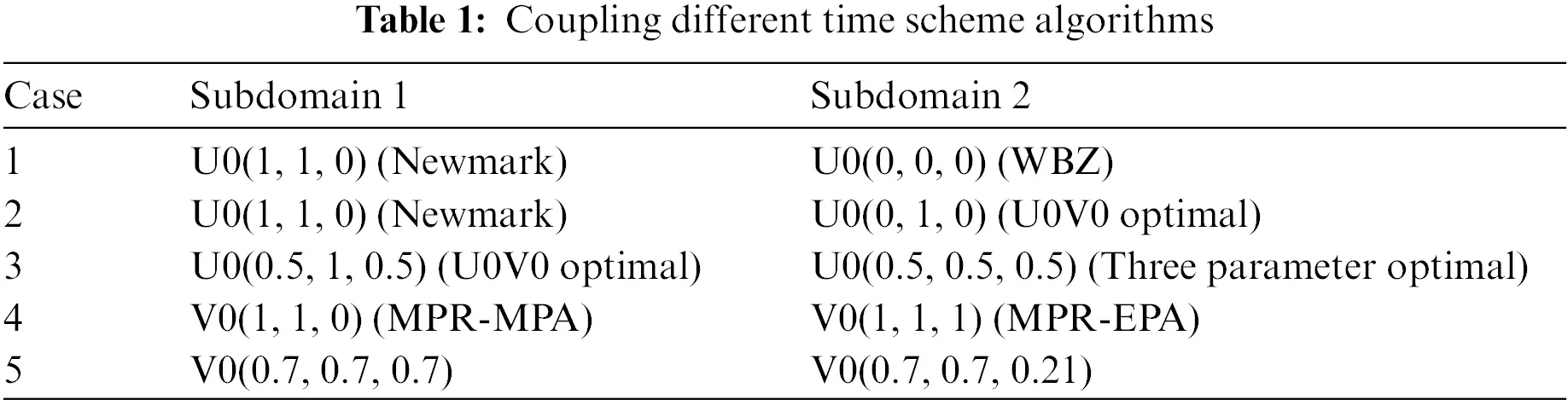For the U0 family of GS4-II algorithms, the W1 value is determined by ρs parameter; whereas, for the V0 family, ρmin and ρmax parameters control the W1 value as shown below. It is also possible to couple U0 and V0 family if the W1 condition is satisfied. For example, U0(1, 1, 0) scheme and V0(1/3, 1/3, 1/3) scheme both produce W1 value of 1; thus, they can be coupled together. These criteria must be followed to preserve second-order time accuracy in all the variables and the Lagrange multipliers.

ForGS4-IIU0Family:W1=11+ρs(91)

ForGS4-IIV0andV0Family:W1=3+ρmin+ρmaxρminρmax2(1+ρmin)(1+ρmax)(92)

5  Numerical Examples

5.1 2D Wave Propagation Problem

Fig. 5 shows the 2D geometry of a wave propagation problem. It is a rectangular bar of 10 m by 1 m with 1 m thickness, which is fixed on the left side wall as boundary condition. The semidiscrete equation of motion for the system is given as

Miu..i+Kiui=GiTλi(93)

i=1i=NdomGivi=0(94)

u(0,y,t)=0(95)

ux(L,y,t)=p(96)

u(x,y,0)=0(97)

u.(x,y,0)=0(98)Figure 5: Diagram of structure for wave propagation problem

The bar is compressed from the right side with p, the distributed load, of 10,000 N/m. This material has 72 GPa for Young’s modulus, 0.3 for Poisson’s ratio, and 2712kg/m3 for density. The bar is divided into two subdomains, and each subdomain is implemented with 17 nodes/particles in length, and 5 nodes/particles in height making it total of 170 nodes/particles with 5 nodes/particles overlap on the interface. Figs. 6 and 7 show the FEM mesh and particle distribution for the two subdomains. The system Δt for time integration is set to be 0.8 μs. The time history of the x-direction displacement, velocity and acceleration of a node/particle at the interface on the subdomain 1 (SD1) side (point A in Fig. 5) and the free end on the subdomain 2 (SD2) side (point B in Fig. 5) are evaluated. We compare the result in four sets: spatial method variance where we combine the different spatial methods; explicit and implicit variance where we pair explcit and implicit version of the same time scheme; time shceme variance where the different combination of the GS4-2 time schemes are tested; and time subcycling variance.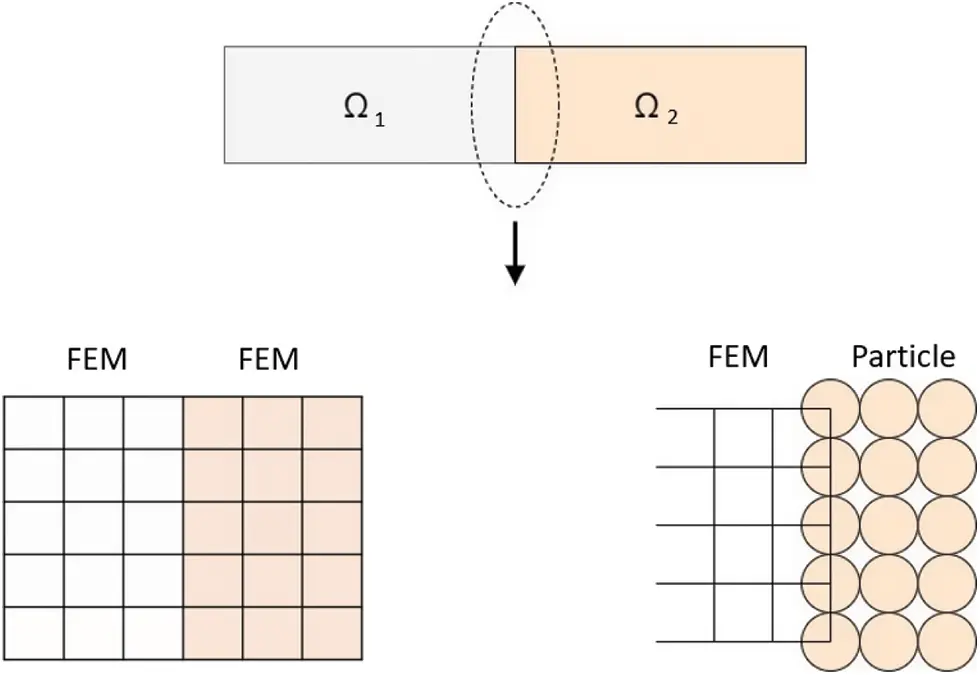Figure 6: FEM+FEM and FEM+GPS interface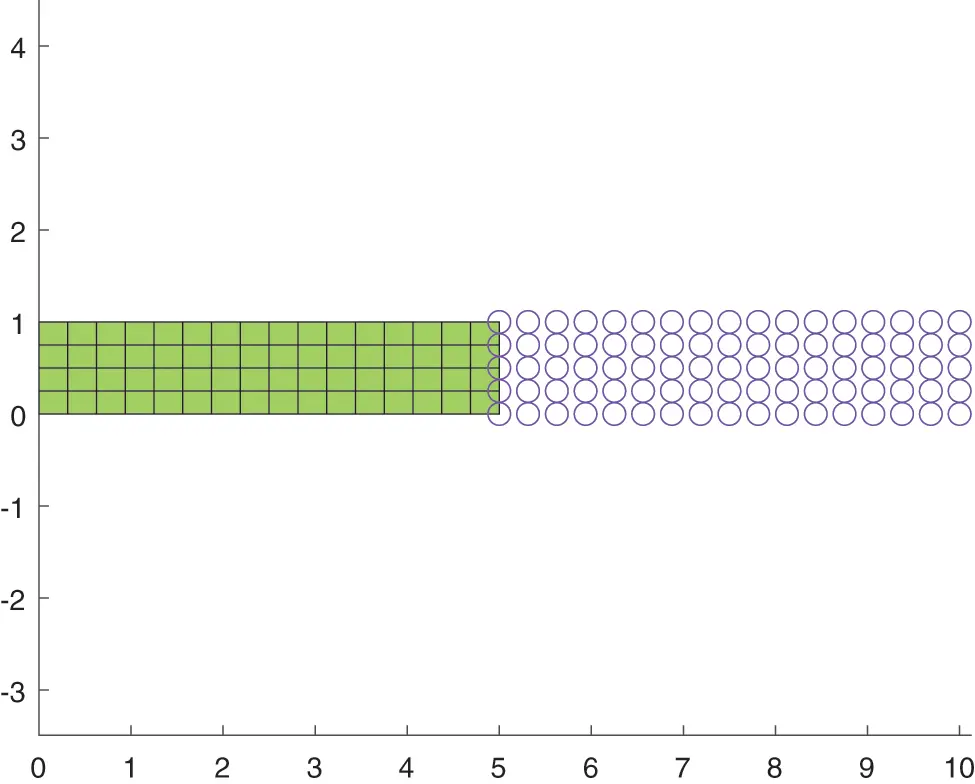Figure 7: FEM+GPS two domain mesh of the structure

Fig. 8 compares the three different cases of coupling different methods: FEM+FEM, GPS+GPS, and FEM+GPS; and shows the time history of the displacement, velocity, and acceleration at A in subdomain 1 and at point B in subdomain 2. It is clear that coupling the same methods or coupling different methods, be it weak form or strong form methods together, produce a comparable result with the result from commercial software such as ABAQUS.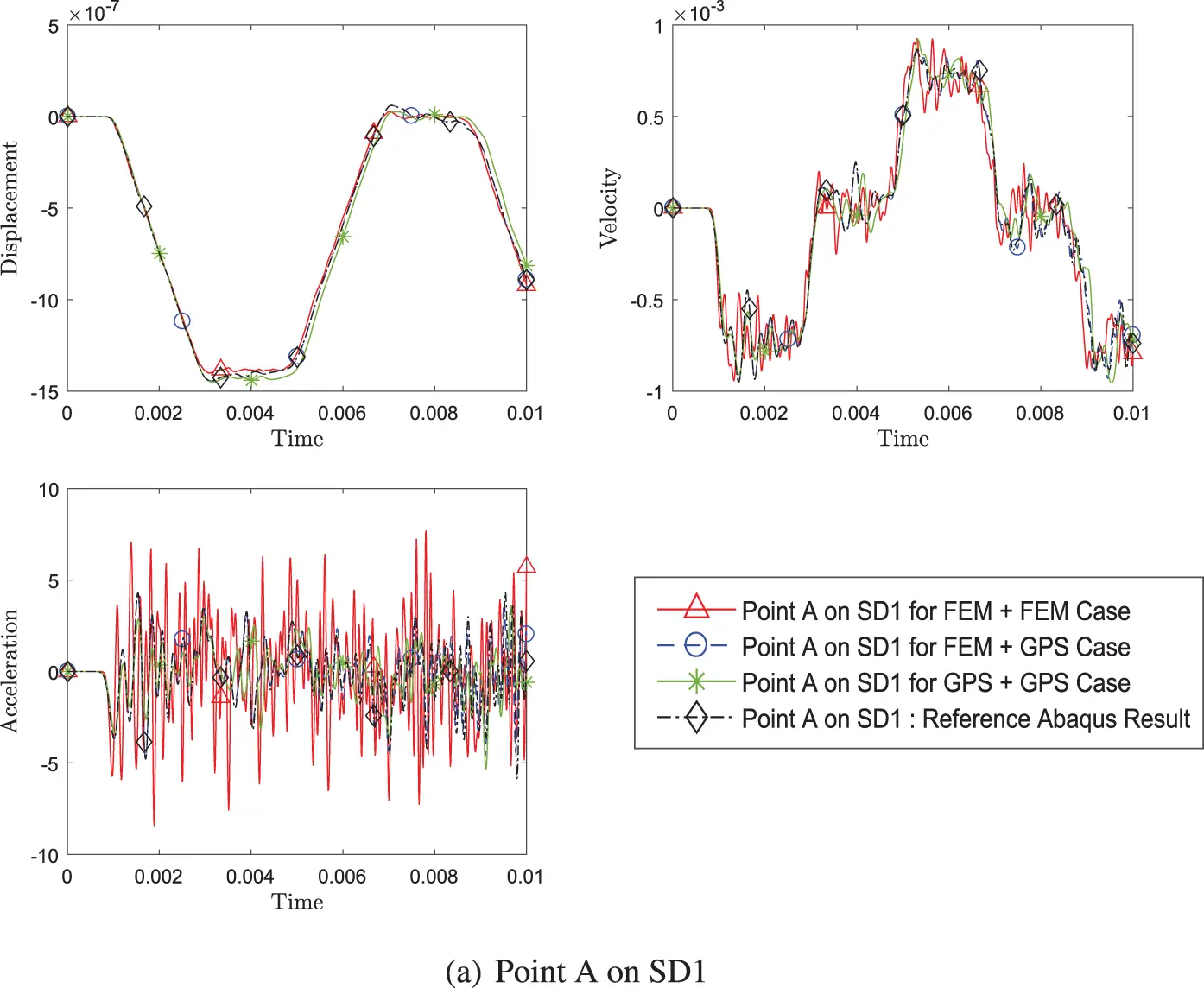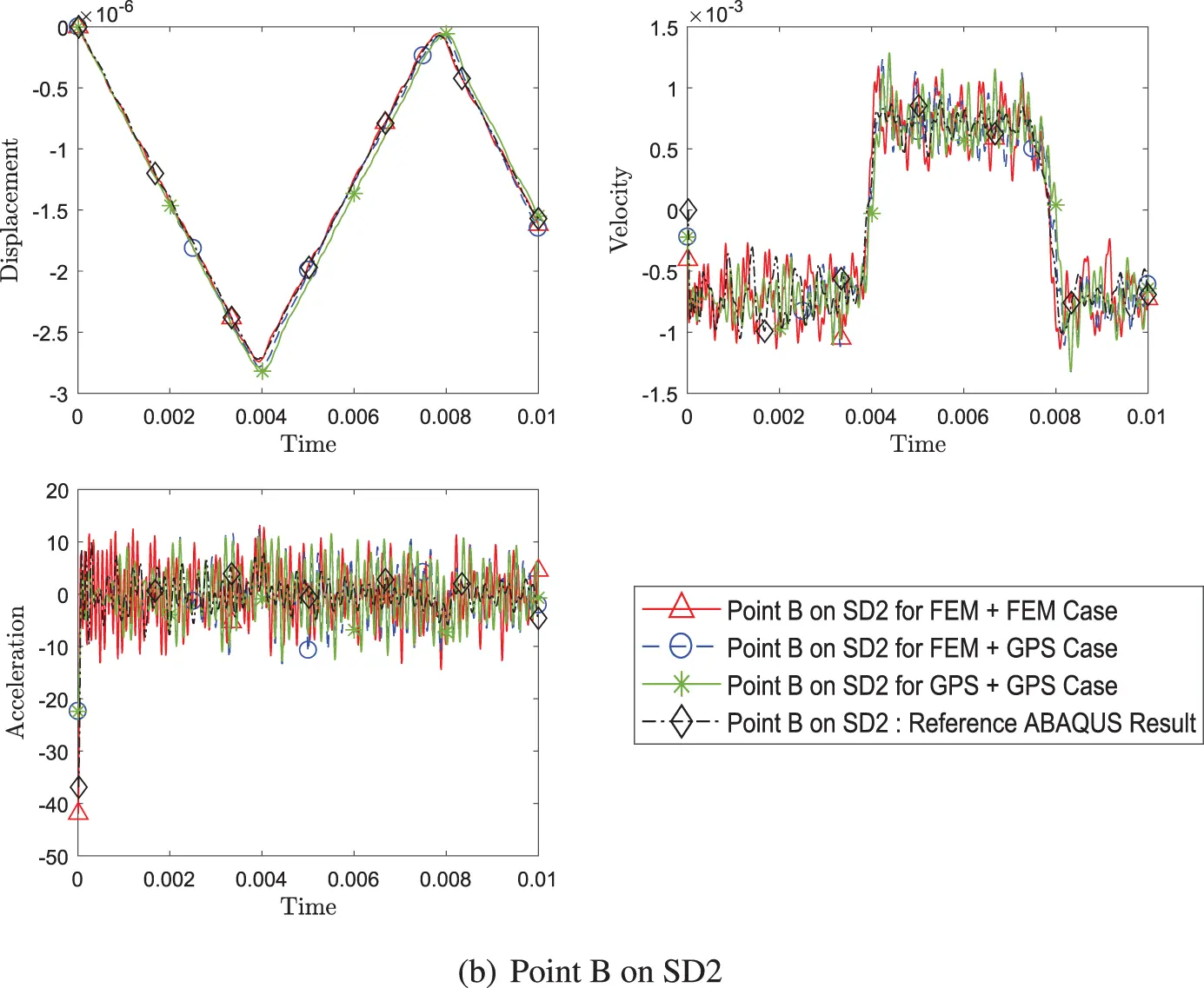Figure 8: Comparison of transient response of displacement, velocity and acceleration (a) at point A on SD1 and (b) at point B on SD2, between three cases of different pairing of methods (Case 1: FEM+FEM, Case 2: FEM+GPS, Case 3: GPS+GPS) while using explicit version (with η3 = 0) of GS4-II U0(1, 1, 0) algorithm for subomain 1 and explicit version (with η3 = 0) of U0(0, 0, 0) algorithm for subdomain 2 with constant Δt in each case

Fig. 9 shows the result for coupling explicit version and implicit version of the schemes. The evaluated coupling cases are explicit and explicit case, explicit and implicit case, and implicit and explicit case where the first condition refers to the time scheme algorithm for subdomain 1 and the latter for subdomain 2. This figure not only suggests that explicit and implicit time algorithms can be coupled together, it also shows that coupling is not limited to just Newmark family of algorithms as this example couples U0(1, 1, 0) (equivalent to Newmark, central difference for explicit, and average acceleration for implicit) and U0(0, 0, 0) (equivalent to WBZ).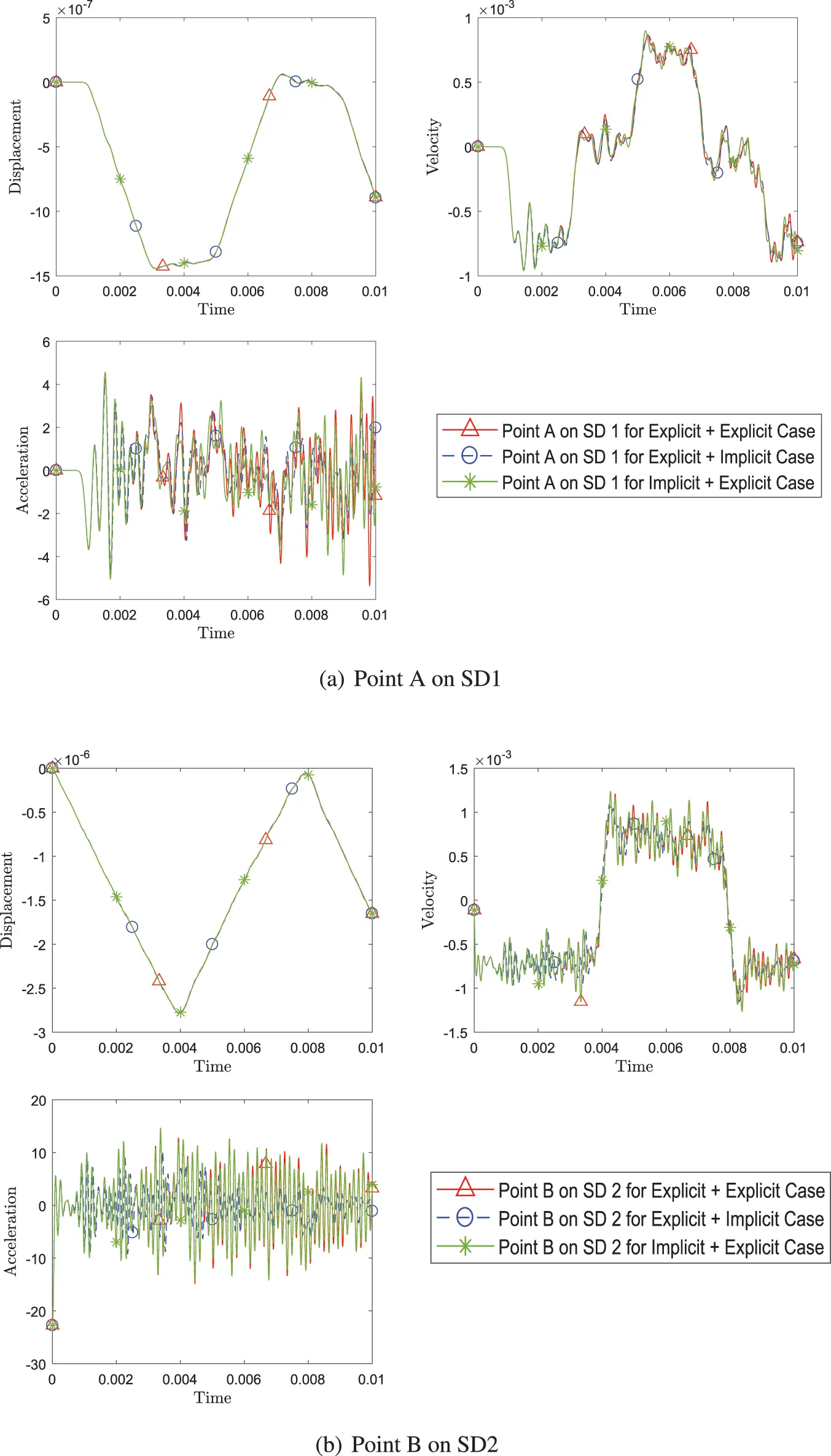Figure 9: Comparison of transient response of displacement, velocity and acceleration (a) at point A on SD1 and (b) at point B between different pairing of explicit (with η3 = 0) and implicit version (with η3 = 1) of GS4-II (Case 1: explicit+explicit, Case 2: explicit+implicit, Case 3: implicit+explicit) while using FEM with GS4-II U0(1, 1, 0) algorithm for subomain 1 and GPS with U0(0, 0, 0) algorithm for subdomain 2 at constant Δt in each case

To further present evidence of coupling various time scheme algorithms, five different cases listed in Table 1, are evaluated and illustrated in Fig. 10. The time scheme algorithms are selected such that a combination has identical W1 to ensure the second order accuracy in time. It is evident that all the results align closely.Figure 10: Comparison of transient response of displacement, velocity and acceleration (a) at point A on SD1 and (b) at point B between different pairing of explicit version (with η3 = 0) of GS4-II algorithms listed in Table 1, while using FEM for subdomain 1 and GPS for subdomain 2 at constant Δt in each case

Lastly, the subcycling cases are also evaluated in Fig. 11. A case where subdomain 2 has double the time steps than subdomain 1 (time increment interval in subdomain 2 is half of that of subdomain 1) is compared with the no subcycling case and the results are very close.Figure 11: Comparison of transient response of displacement, velocity and acceleration at both point A and point B between simulation with system Δt for both subdomains and with variable Δt = 1/2 system Δt for subdomain 2 while using FEM with explicit version (with η3 = 0) of GS4-II U0(1, 1, 0) algorithm for subdomain 1 and GPS with explicit version (with η3 = 0) U0(0, 0, 0) algorithm for subdomain 2

Fig. 12 demonstrates second order time accuracy in displacement, velocity, acceleration and the constraint variable λ for different coupling conditions shown through Figs. 810.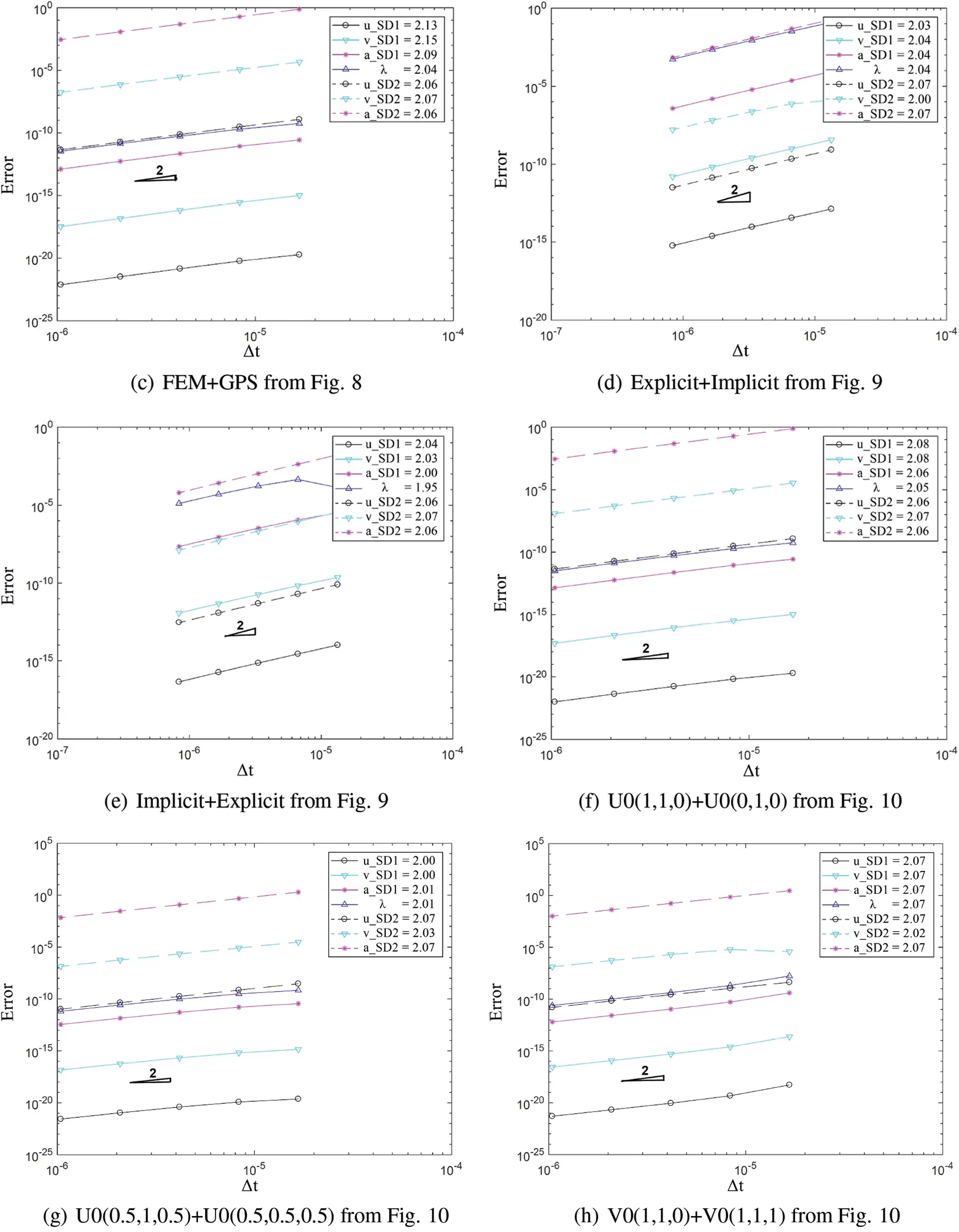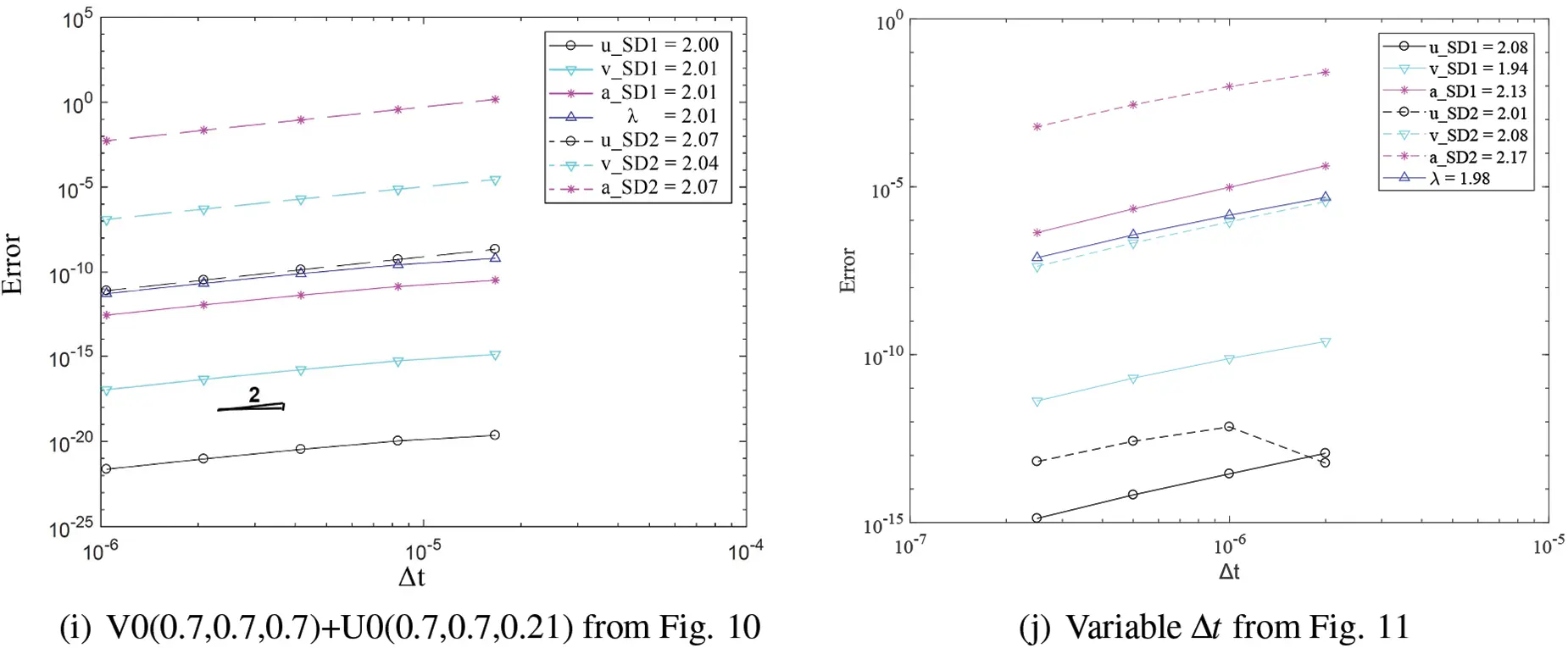Figure 12: Convergence order for the solution with respect to the system time step for domain 1 and 2 in different cases: (a) FEM with explicit version of GS4-II U0(1, 1, 0) and FEM with U0(0, 0, 0), (b) GPS with explicit version of GS4-II U0(1, 1, 0) and GPS with U0(0, 0, 0), (c) FEM with explicit version of GS4-II U0(1, 1, 0) and GPS with U0(0, 0, 0) algorithm, (d) FEM with explicit version of GS4-II U0(1, 1, 0) and GPS with implicit version U0(0, 0, 0), (e) FEM with implicit version of GS4-II U0(1, 1, 0) and GPS with explicit version U0(0, 0, 0), (f) FEM with explicit version of GS4-II U0(1, 1, 0) and FEM with implicit version U0(0, 1, 0), (g) FEM with explicit version of GS4-II U0(0.5, 1, 0.5) and GPS with U0(0.5, 0.5, 0.5), (h) FEM with explicit version of GS4-II V0(1, 1, 0) and GPS with V0(1, 1, 1), (i) FEM with explicit version of GS4-II V0(0.7, 0.7, 0.7) and GPS with V0(0.7, 0.7, 0.21), (j) variable Δt = 1/2 system Δt for subdomain 2 while using FEM with explicit version (with η3 = 0) of GS4-II U0(1, 1, 0) algorithm for subdomain 1 and GPS with explicit version (with η3 = 0) U0(0, 0, 0) algorithm for subdomain 2

5.2 2D Vibration Problem

A beam with the same geometry as the one in the wave propagation problem is used to illustrate the application of the multi-domain method in a plane stress dynamic problem. A cantilever beam of 10 m by 1 m with 1 m thickness is fixed on the left side and is experiencing downward traction, p, of 100,000 N/m as shown in Fig. 13. The density, ρ, of the beam is set to be 271.2kg/m3, Young’s modulus, E, to be 72 GPa, and Poisson’s ratio to be 0.3 for the properties of the material. The beam is divided into two subdomains, and each subdomain is implemented with 17 nodes/particles in length, and 5 nodes/particles in height making it total of 170 nodes/particles with 5 nodes/particles overlap on interface. The semidiscrete equation of motion for the system is given as

Miu..i+Kiui=GiTλi(99)

i=1i=NdomGivi=0(100)

u(0,y,t)=0(101)

uy(L,y,t)=p(102)

u(x,y,0)=0(103)

u.(x,y,0)=0(104)Figure 13: Diagram of structure for bending problem

The system Δt for time integration is set to be 0.8 μs. The time history of y-direction displacement, velocity and acceleration of a node at the interface (point A) and the free end (point B) are evaluated.

Similar to the previous problem, a different combination of methods are compared in Fig. 14. It is apparent that the different methods can be coupled regardless of whether one is weak form or strong form.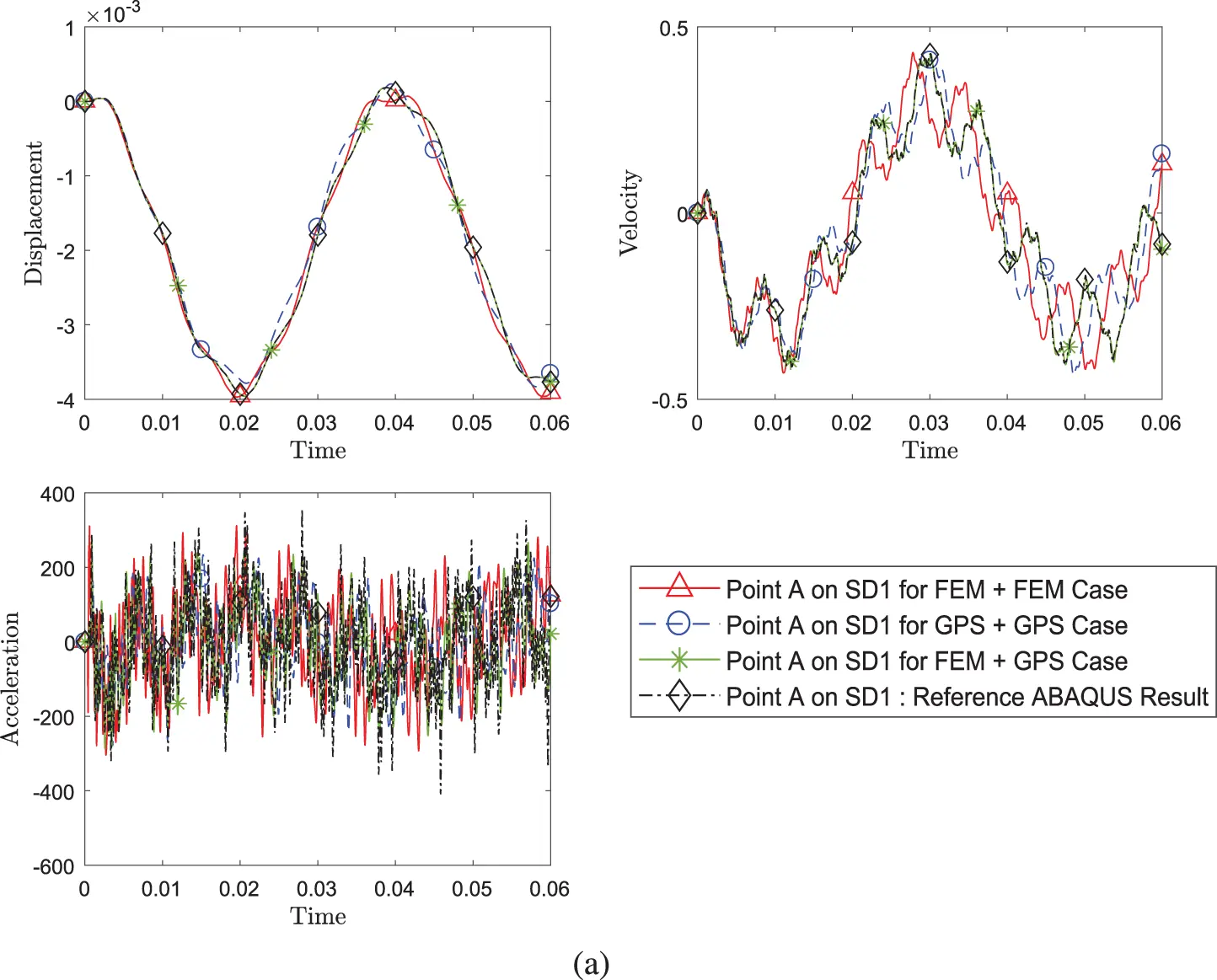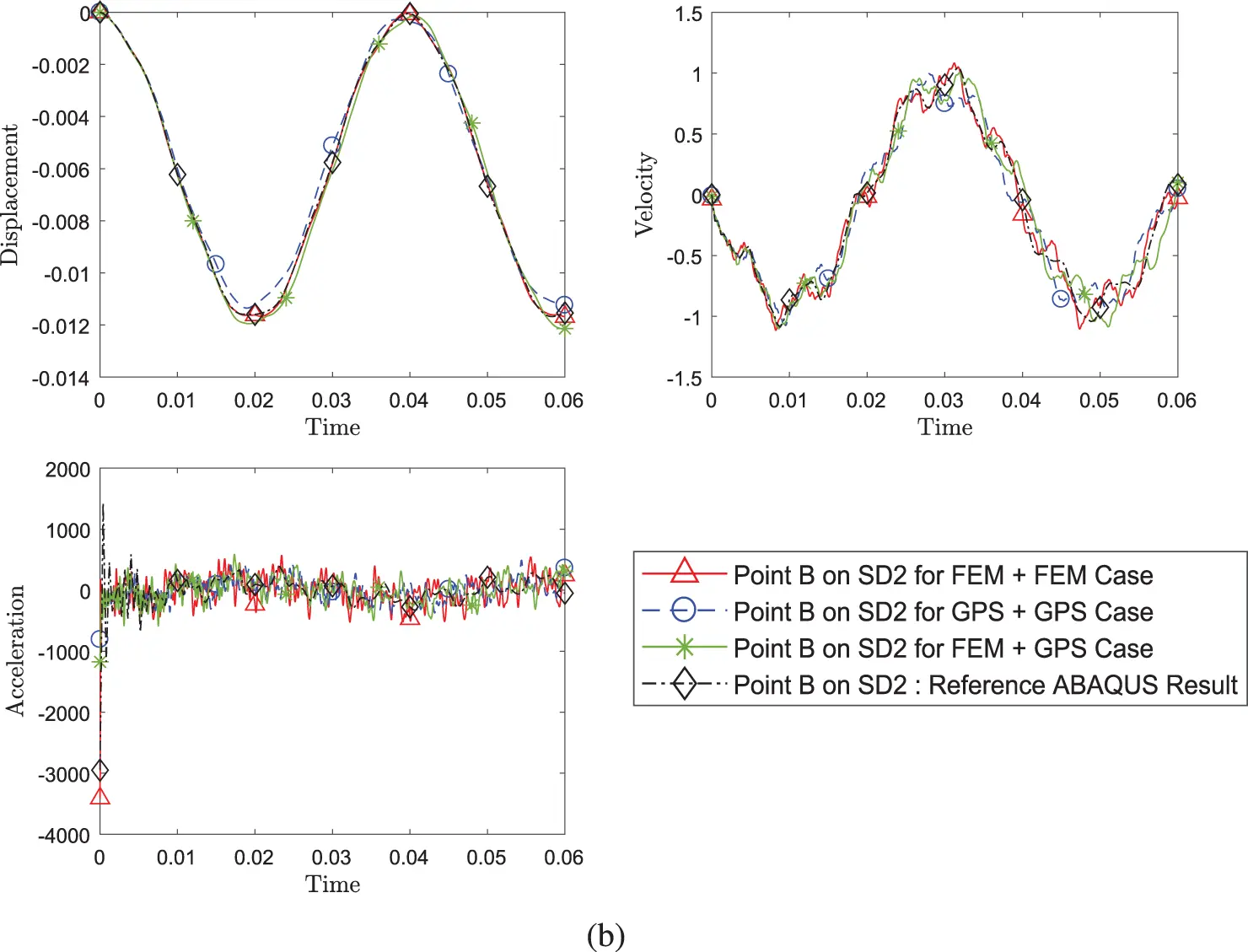Figure 14: Comparison of transient response of displacement, velocity and acceleration (a) at point A on SD1 and (b) at point B between different pairing of methods (Case 1: FEM+FEM, Case 2: FEM+GPS, Case 3: GPS+GPS) while using implicit version (with η3 = 1) of GS4-II U0(1, 1, 0) algorithm for subdomain 1 and implicit version of U0(0, 0, 0) algorithm for subdomain 2 with constant Δt in each case

Fig. 15 shows results for coupling the explicit version and implicit version of the time scheme algorithms. The evaluated coupling cases this time are the implicit and implicit case, explicit and implicit case, and implicit and explicit case. The response results for the different cases align closely. The same five cases of time scheme algorithm combinations used in the previous example are evaluated and illustrated in Fig. 16. And the results for all cases match. The subcycling case with one domain having half the Δt as the other, is shown in Fig. 17. It is apparent that subcycling still produces an accurate result. Lastly, all of the different cases and conditions are second order accurate in time as evident from Fig. 18, illustrating slope of two in the log-log error convergence plot.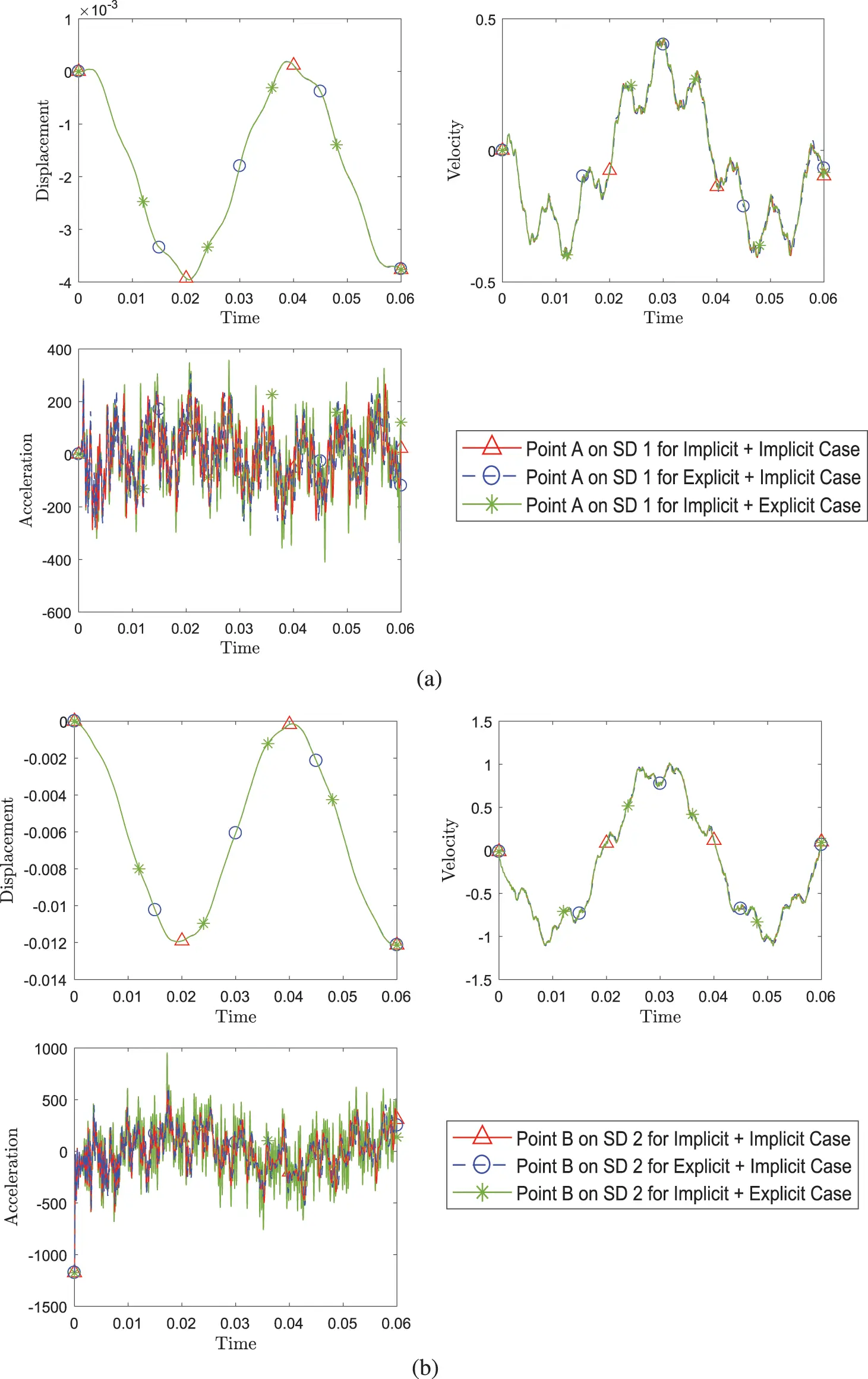Figure 15: Comparison of transient response of displacement, velocity and acceleration (a) at point A on SD1 and (b) at point B between different pairing of explicit (with η3 = 0) and implicit version (with η3 = 1) of GS4-II (Case 1: implicit+implicit, Case 2: explicit+implicit, Case 3: implicit+implicit) while using FEM with GS4-II U0(1, 1, 0) algorithm for subdomain 1 and GPS with U0(0, 0, 0) algorithm for subdomain 2 at constant Δt in each case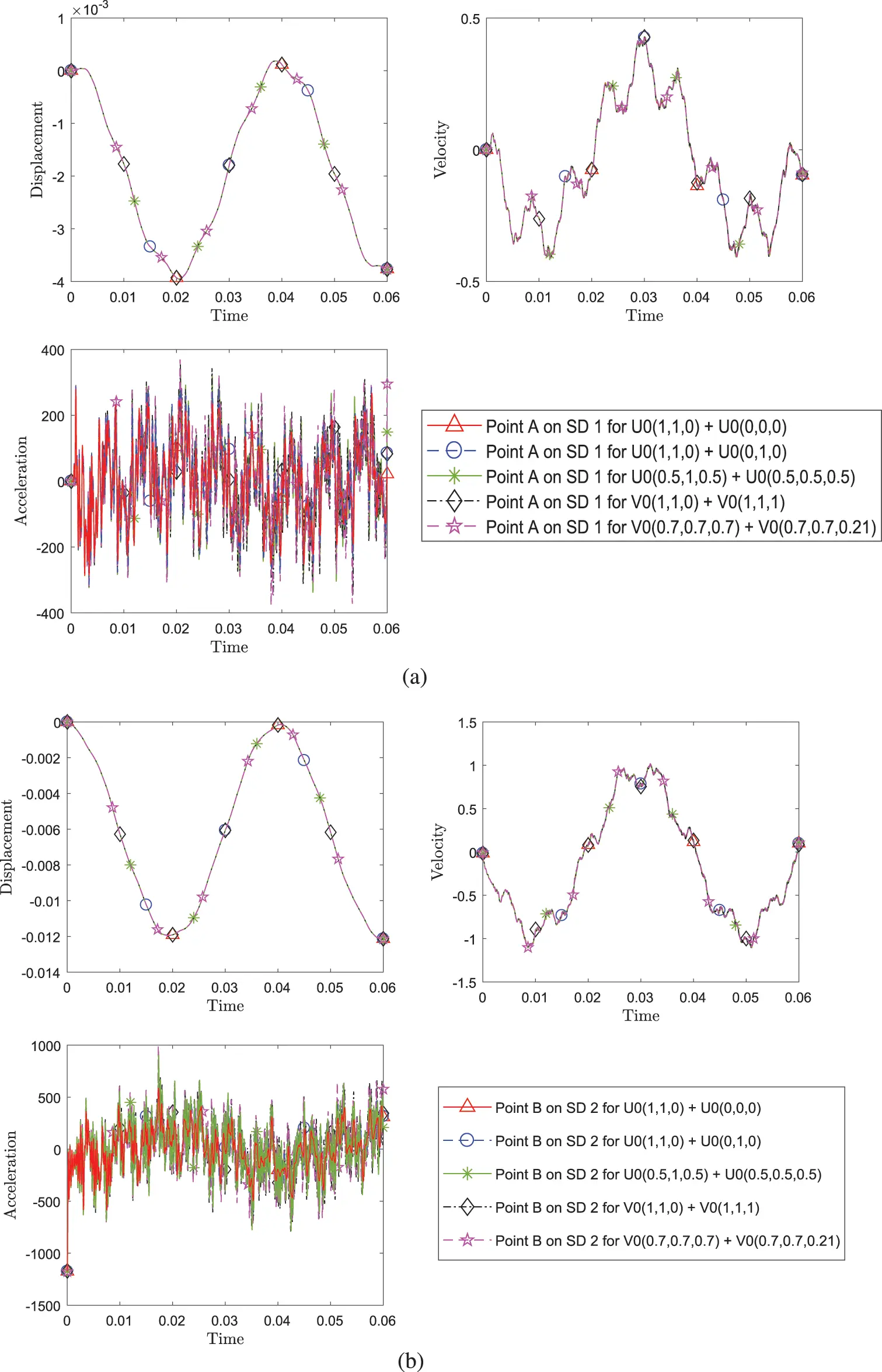Figure 16: Comparison of transient response of displacement, velocity and acceleration (a) at point A on SD1 and (b) at point B between different pairing of implicit version (with η3 = 1) of GS4-II algorithms listed in Table 1 while using FEM for subdomain 1 and GPS for subdomain 2 at constant Δt in each case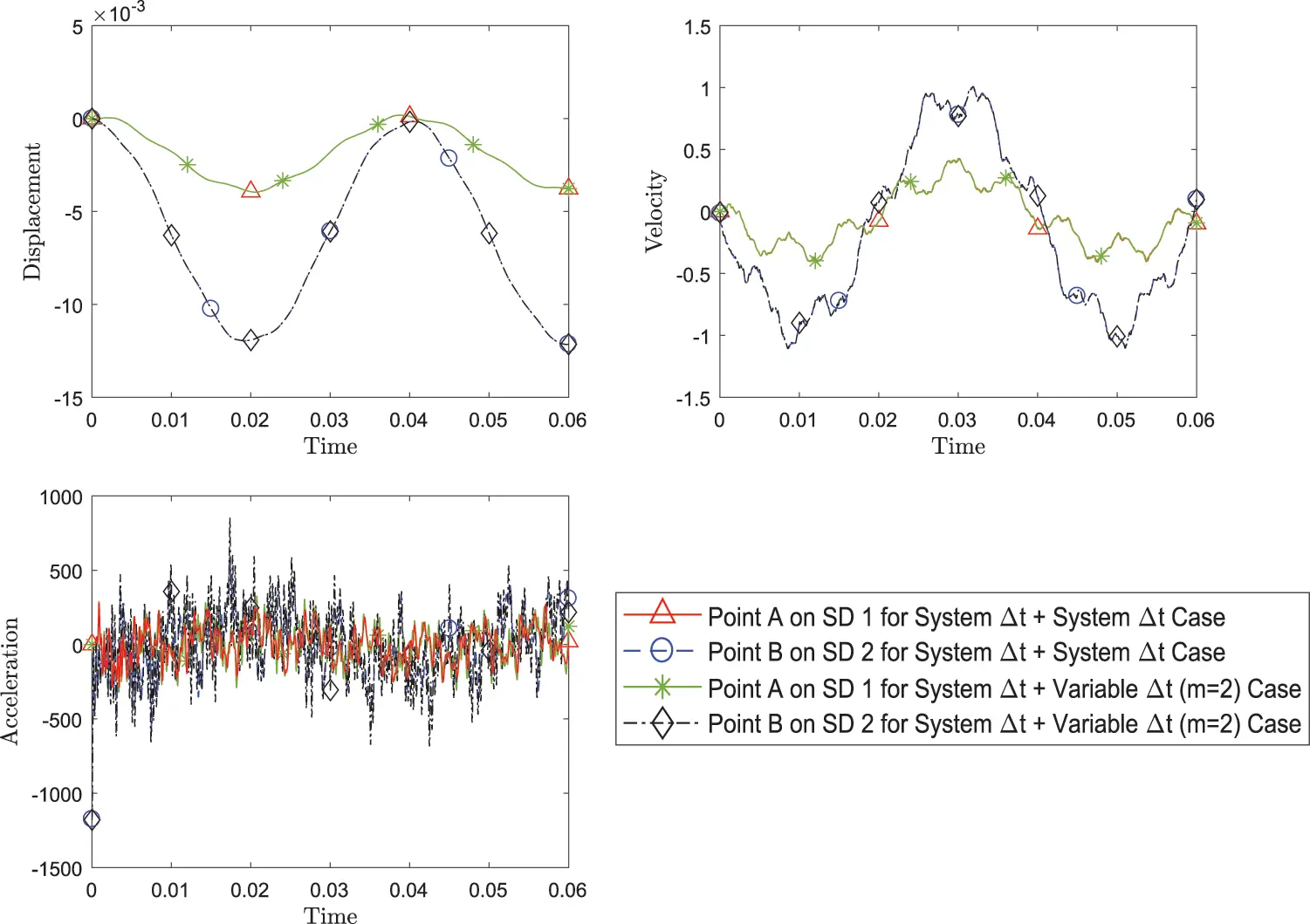Figure 17: Comparison of transient response of displacement, velocity and acceleration at both point A and point B, between simulation with system Δt for both subdomains and with variable Δt = 1/2 system Δt for subdomain 2 while using FEM with implicit version (with η3 = 1) of GS4-II U0(1, 1, 0) algorithm for subdomain 1 and GPS with implicit version (with η3 = 1) U0(0, 0, 0) algorithm for subdomain 2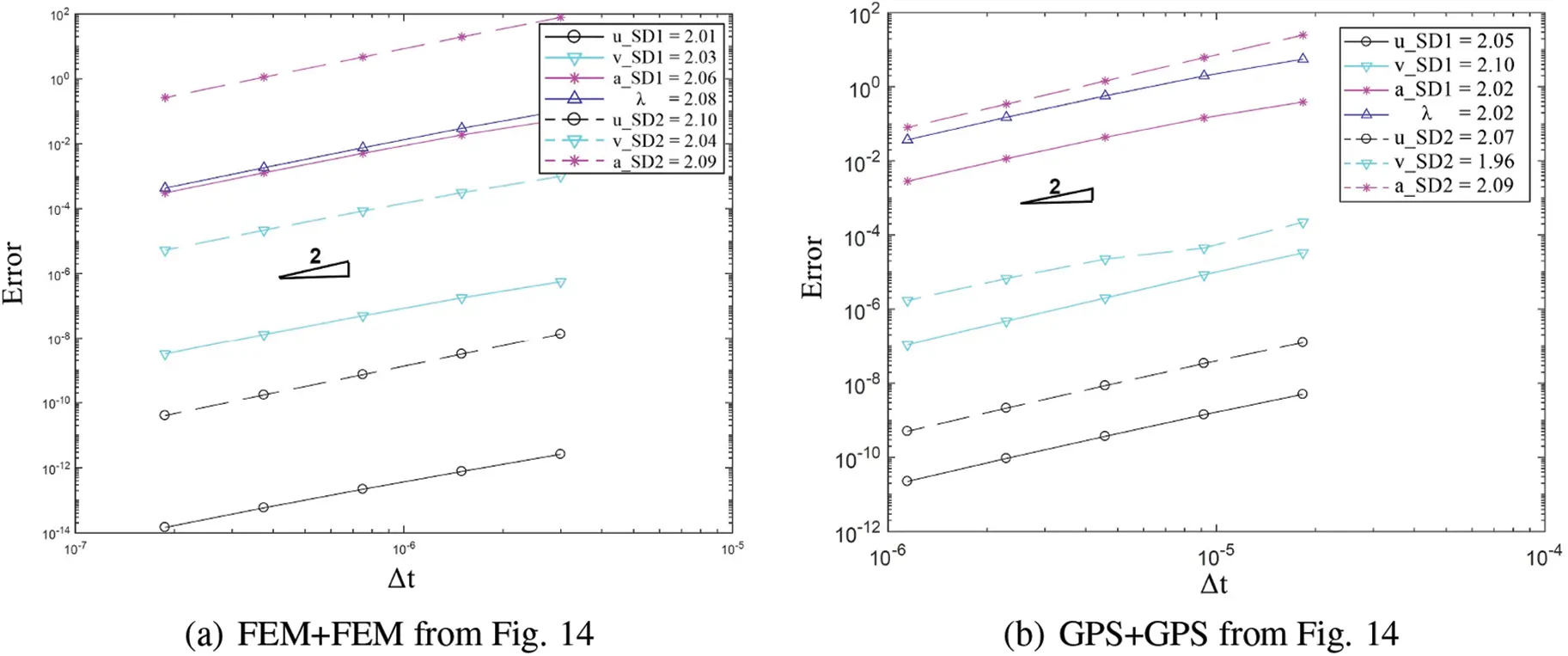Figure 18: Convergence order for the solution with respect to the system time step for domain 1 and 2 in different cases: (a) FEM with implicit version of GS4-II U0(1, 1, 0) and FEM with U0(0, 0, 0), (b) GPS with implicit version of GS4-II U0(1, 1, 0) and GPS with U0(0, 0, 0), (c) FEM with implicit version of GS4- II U0(1, 1, 0) and GPS with U0(0, 0, 0), (d) FEM with explicit version of GS4-II U0(1, 1, 0) and GPS with implicit version U0(0, 0, 0), (e) FEM with implicit version of GS4-II U0(1, 1, 0) and GPS with explicit version U0(0, 0, 0), (f) FEM with implicit version of GS4-II U0(1, 1, 0) and FEM with U0(0, 1, 0), (g) FEM with implicit version of GS4-II U0(0.5, 1, 0.5) and GPS with U0(0.5, 0.5, 0.5) algorithm for subdomain 2, (h) FEM with implicit version of GS4-II V0(1, 1, 0) and GPS with V0(1, 1, 1), (i) FEM with implicit version of GS4-II V0(0.7, 0.7, 0.7) and GPS with V0(0.7, 0.7, 0.21), (j) variable Δt2 = 1/2 system Δt for subdomain 2 while using FEM with implicit version (with η3 = 1) of GS4-II U0(1, 1, 0) algorithm for subdomain 1 and GPS with implicit U0(0, 0, 0) algorithm for subdomain 2

5.3 2D Vibration Problem-Three Subdomains

Next, a body divided into three domains is examined next for the extension of the subdomain coupling concept. The same vibration problem above is evaluated again but this time, the beam is divided into three subdomains where L2=L/10. The FEM is used on subdomains 1 and 3 with 22 nodes in length, and 7 nodes in height. GPS is implemented on subdomain 2, with 7 particles in length and 7 particles in height. The time scheme algorithm used in subdomain 1 and 3 is implicit U0(1, 1, 0); and in subdomain 2, U0(0, 0, 0) is used. The time history of y-direction displacement, velocity and acceleration of a node at the free end is evaluated.

Figs. 20 and 21 clearly illustrate that the result at points A and B (shown in Fig. 19a) from the three subdomain case matches closely with the result from the two subdomain case while achieving second order time convergence rate. The high frequency in the acceleration plot for the three domain free end node is caused by the use of non-dissipative U0(1, 1, 0) scheme.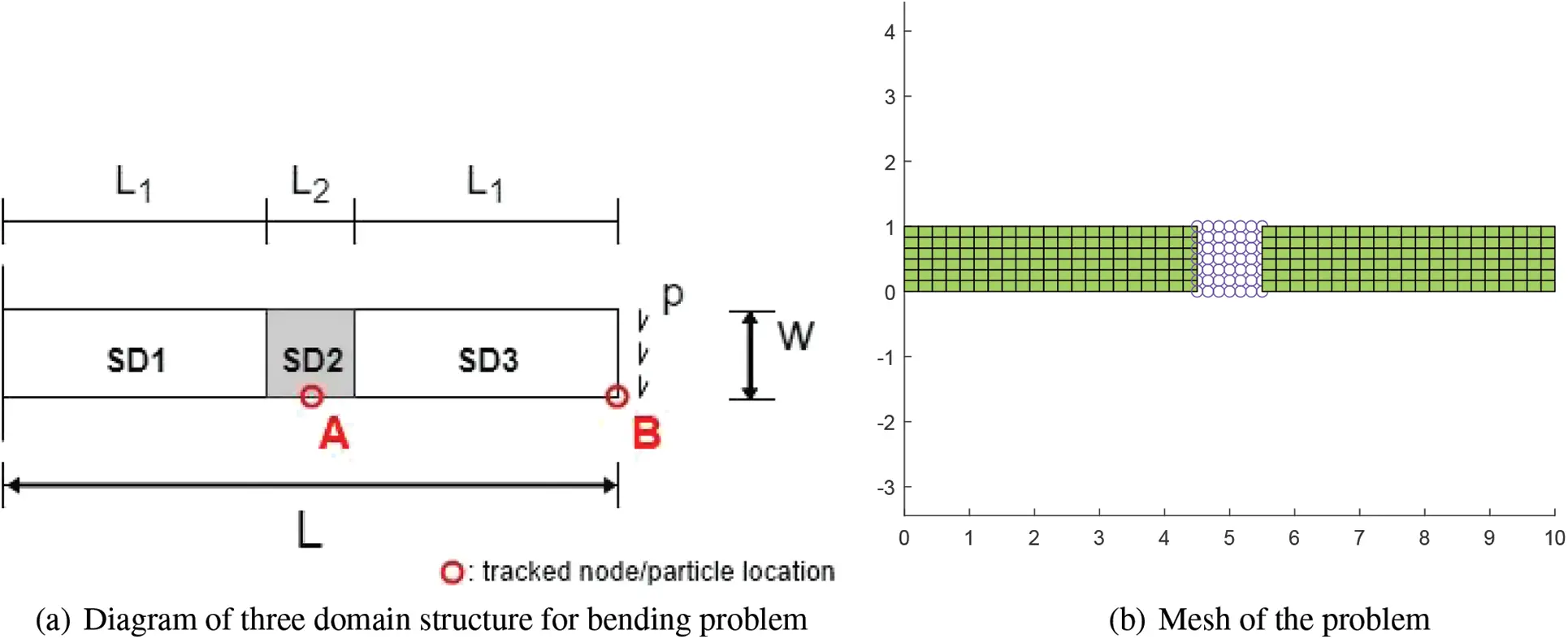Figure 19: (a) Structure divided into three domains (b) An example of mesh of the structure: FEM GPS FEM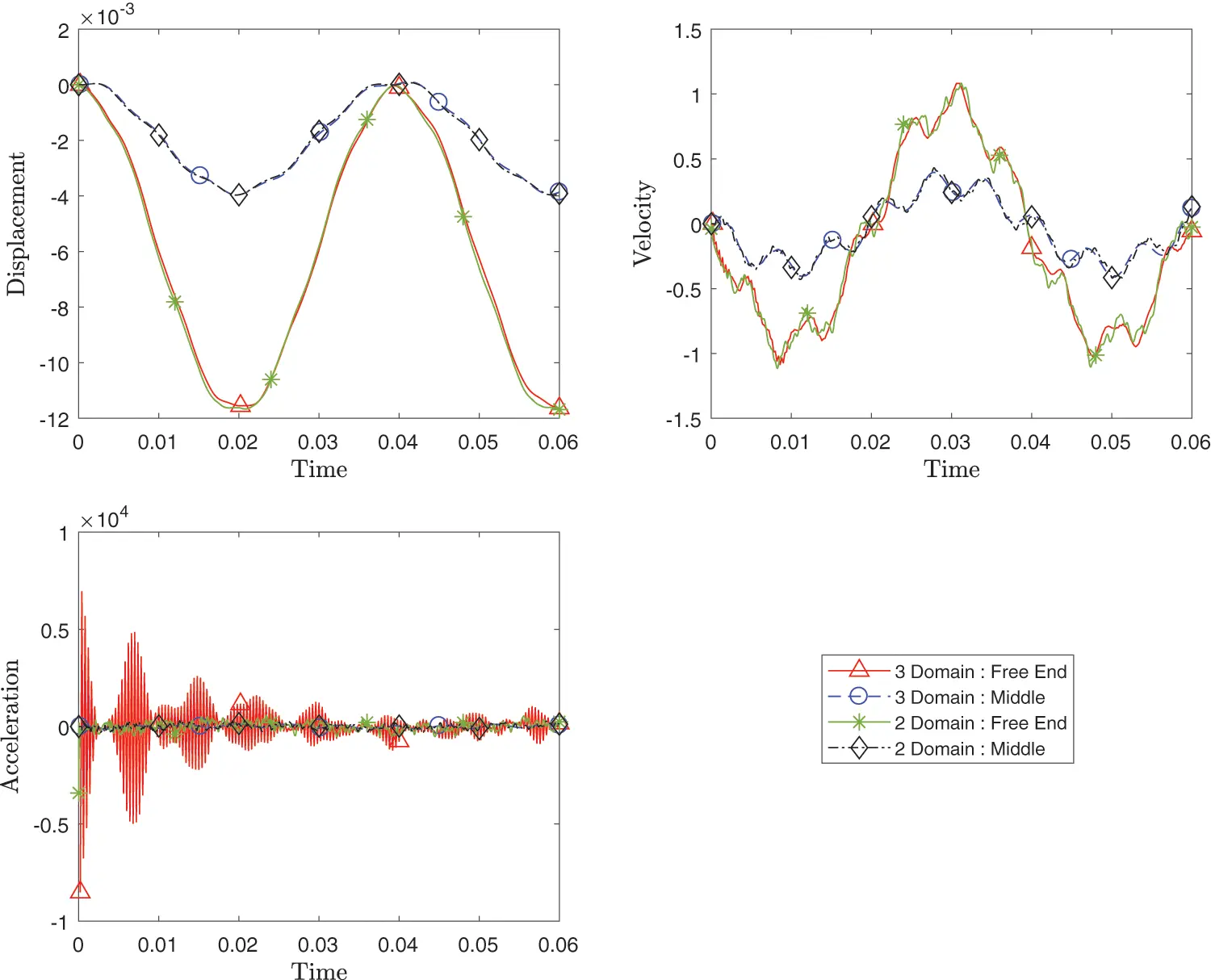Figure 20: Comparison of transient response of displacement, velocity and acceleration at point A and point B between three subdomain configuration and two subdomain configuration while using FEM with implicit version (with η3 = 1) of GS4-II U0(1, 1, 0) algorithm for subdomain 1 and subdomain 3 and GPS with implicit version of U0(0, 0, 0) algorithm for subdomain 2 with constant Δt in all subdomains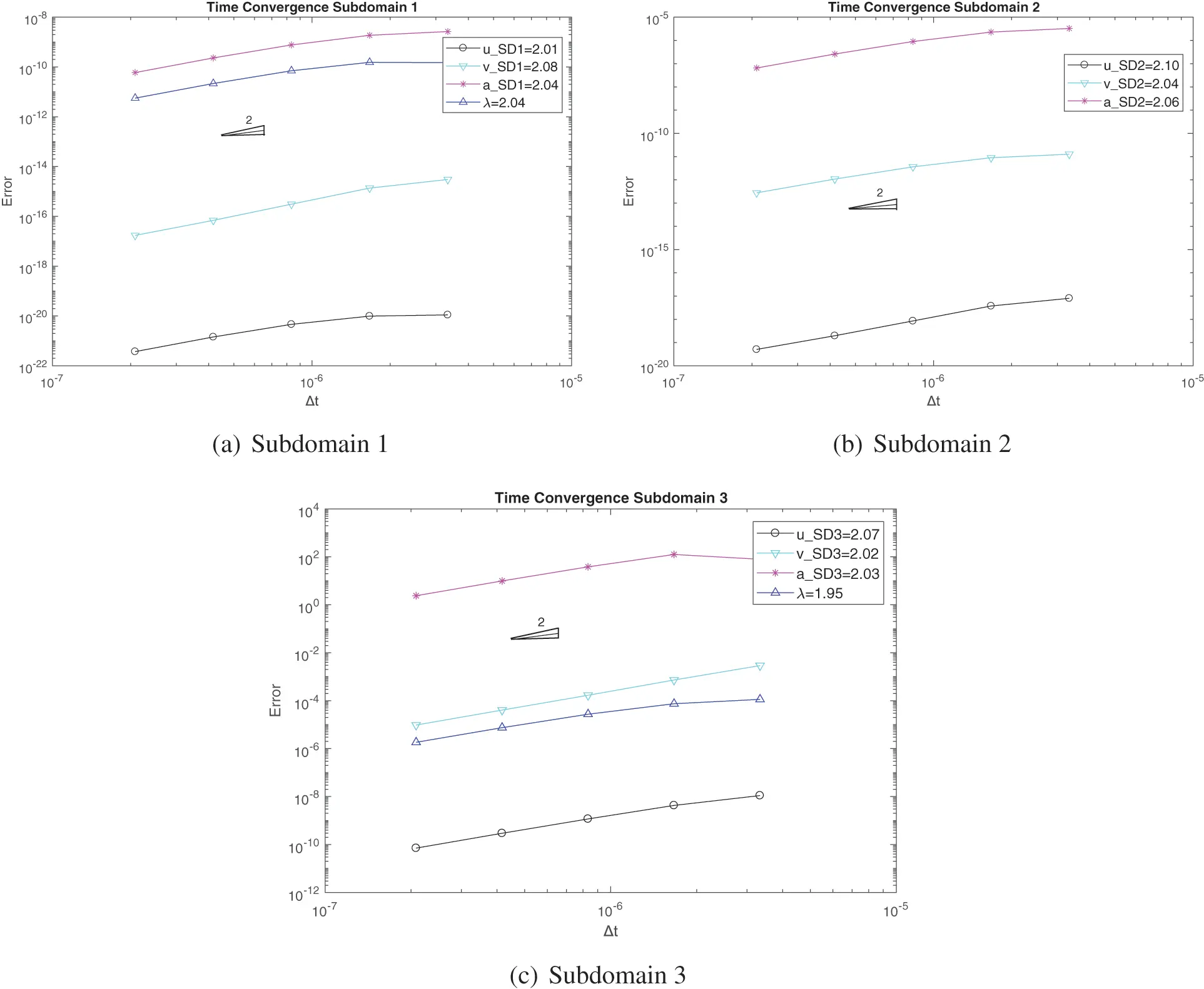Figure 21: Convergence order for the solution with respect to the system time step for domain 1 and 2 and 3 in three domain configuration case: (a) subdomain 1 using FEM with implicit version of GS4-II U0(1, 1, 0) algorithm, (b) subdomain 2 using GPS with U0(0, 0, 0) algorithm, (c) subdomain 3 using FEM with GS4-II U0(1, 1, 0) algorithm

5.4 2D Notched Specimen Problem

Figs. 22 and 23 show the geometry of a 2D plane stress problem and an example of the mesh, respectively. It consists of three subdomains where identical subdomain 1 and 3 are connected by a thin subdomain 2. The subdomain 1 is 3 m by 2 m rectangle which are discretized into 176 FEM nodes. On the other hand, subdomain 2 is 1 m by 2 m rectangle bar, with notches on top and bottom, discretized into 99 particles. And subdomain 3 is identical to the subdomain 1 geometrically, but discretized with 176 particles. The whole geometry has thickness of 1 m. The semidiscrete equation of motion for the system is given as

ρiu..i+Dui=GiTλii=1i=NdomGivi=0u(0,y,t)=0ux(L,y,t)=210u(x,y,0)=0u.(x,y,0)=0(105)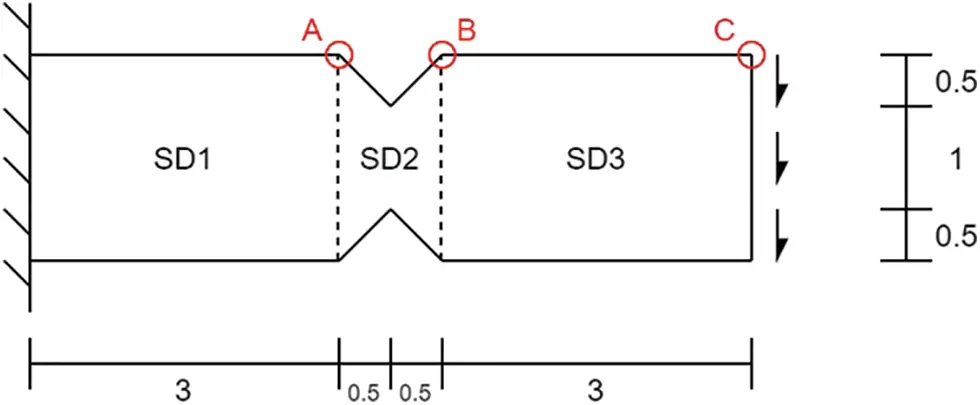Figure 22: Diagram of structure divided into three subdomain. The red circles represent the locations where u, u˙, and ü are tracked in each subdomain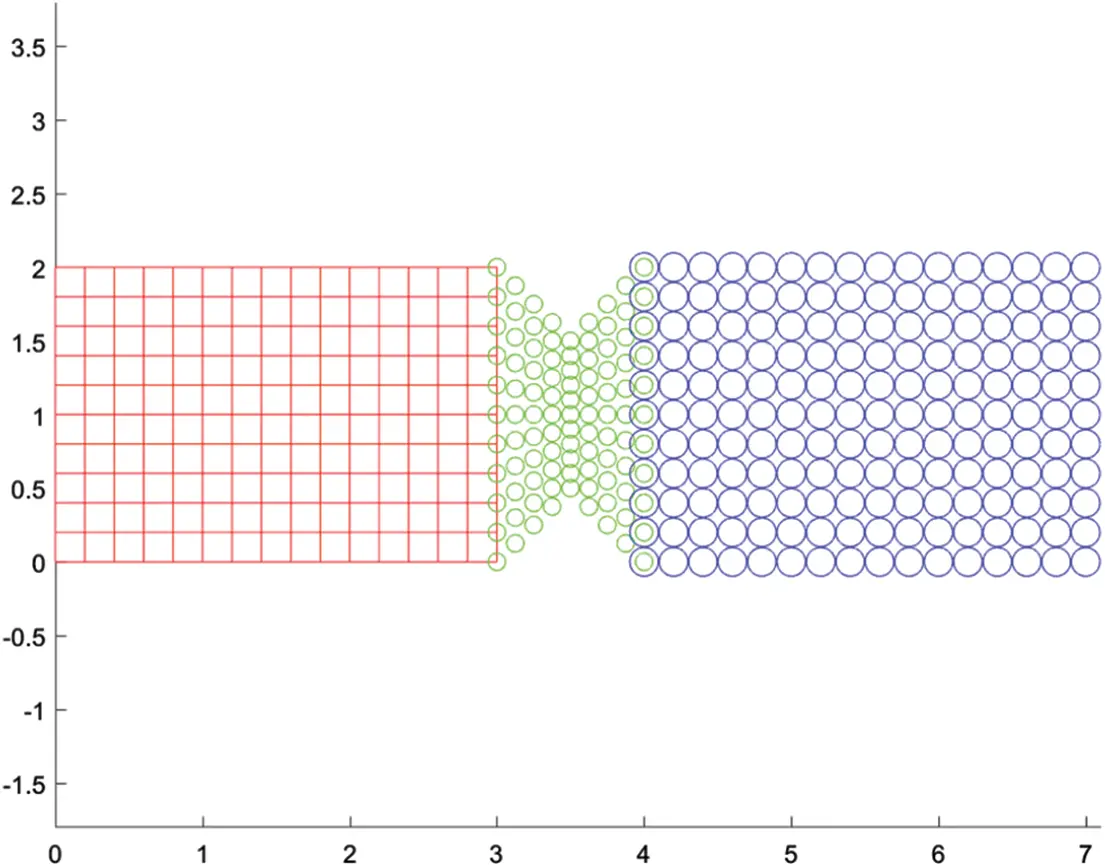Figure 23: Mesh of all three subdomains

The bar is fixed at the left end and pulled down from the right end with a distributed load of 210 N/m. This material has 70 GPa for Young’s modulus, 0.3 for Poisson’s ratio, and 1,000kg/m3 for density. The simulation ran for 0.2 s with the system Δt for time integration set to 0.4 ms. To demonstrate the coupling of different spatial methods and different time schemes, four cases are studied as shown in Table 2.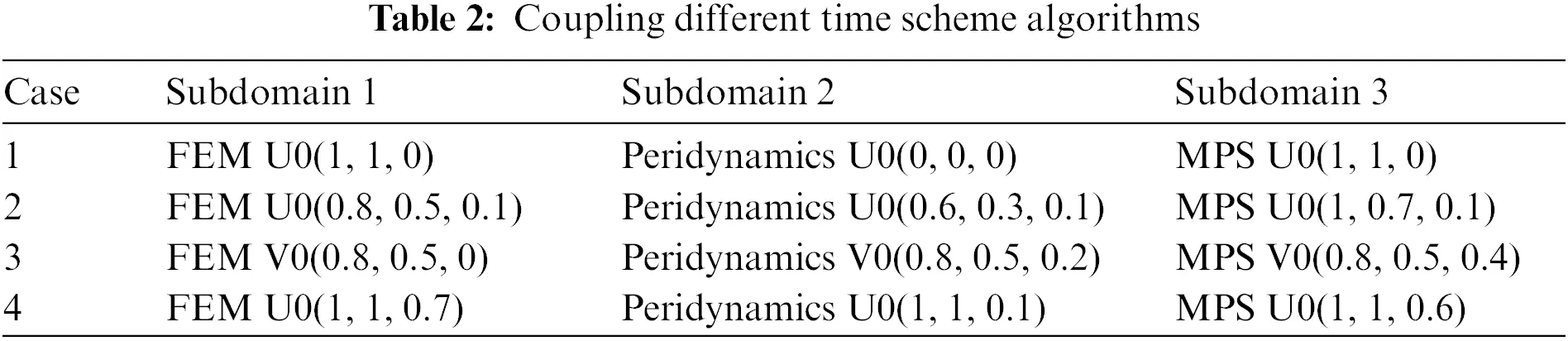The time history of the y-direction displacement, velocity and acceleration of nodes/particles in each subdomain (points A, B, and C in Fig. 22) are evaluated.

Figs. 2426 show the transient response of the displacement, velocity and acceleration for points A, B and C for all cases listed in the Table 2 compared with the reference results. All responses from the different spatial methods and different time schemes combinations match well.Figure 24: Comparison of transient response of displacement, velocity and acceleration at point A between different cases with varying method and time integration schemes for each domain as listed in Table 2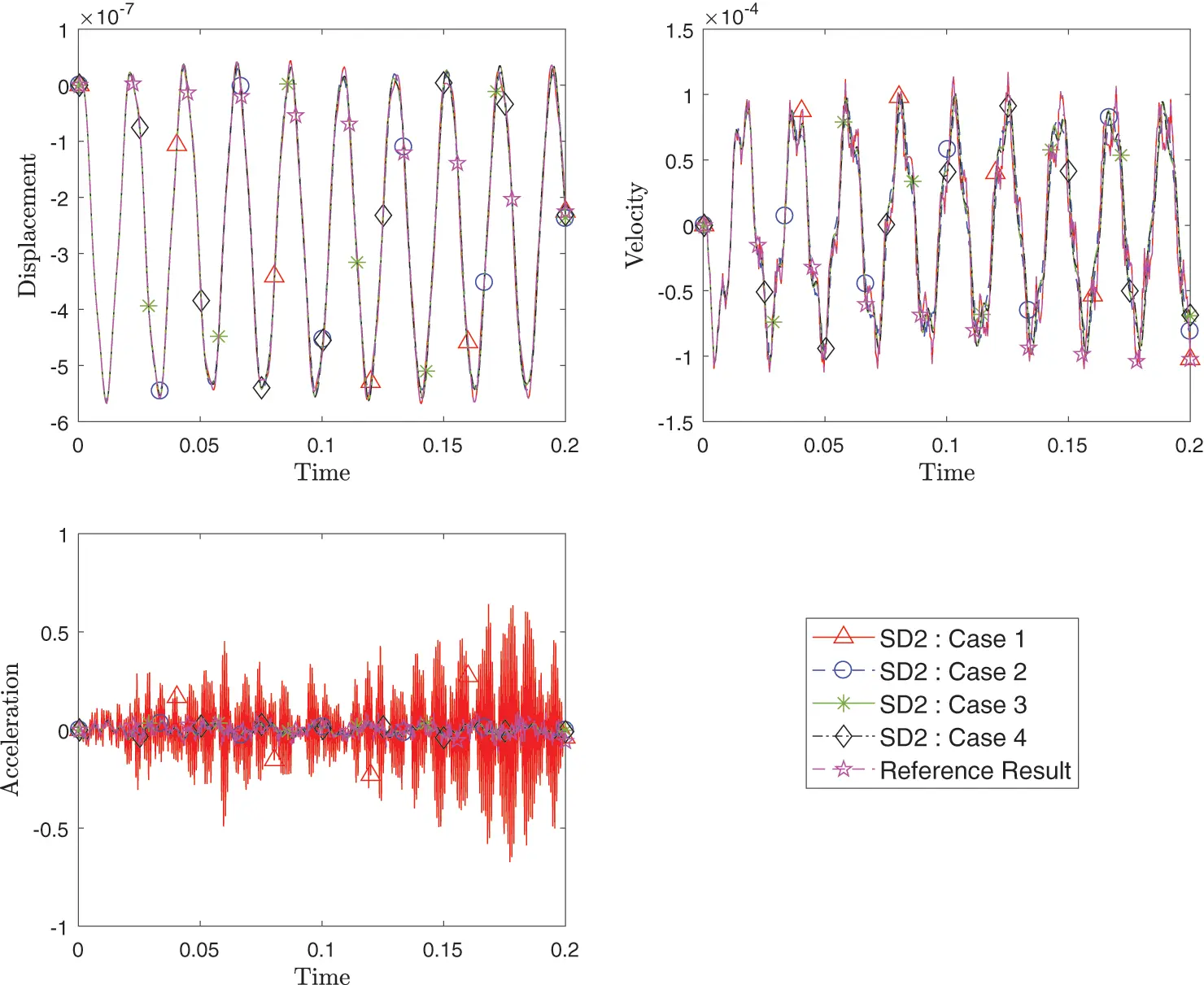Figure 25: Comparison of transient response of displacement, velocity and acceleration at point B among different cases with varying method and time integration schemes for each domain as listed in Table 2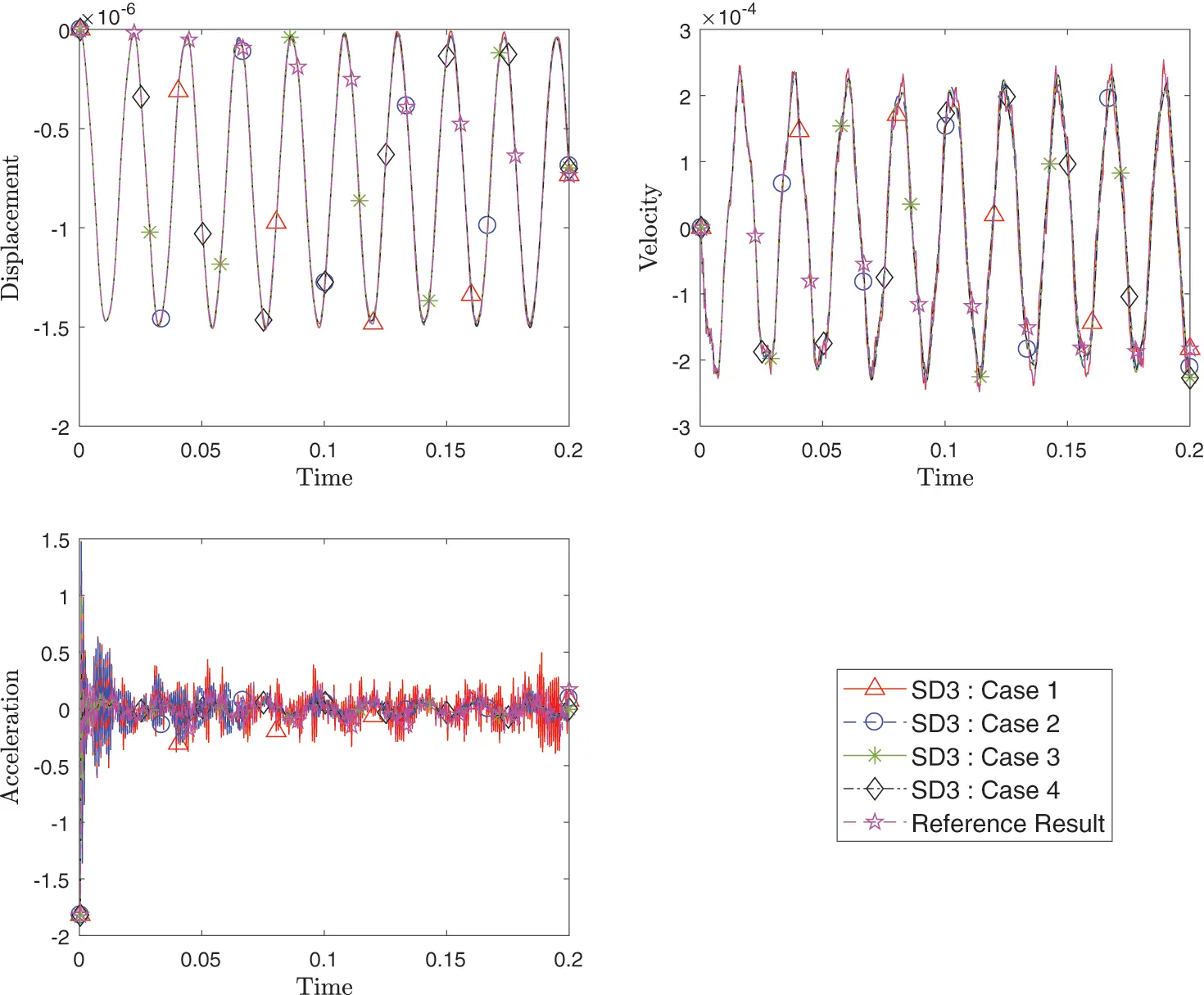Figure 26: Comparison of transient response of displacement, velocity and acceleration at point C among different cases with varying method and time integration schemes for each domain as listed in Table 2

6  Conclusions

In this paper, we have proposed a novel implementation of the Generalized Single Step Single Solve time integration framework for ODE’s into the DAE framework. This proposed method, unlike traditional approaches in current technology with LMS methods, allows the coupling of different numerical analysis methods such as FEM and particle methods to be used on a single body while ensuring the accuracy of the result with the use of a wide variety of time integration algorithms within the GS4 framework. For example, one cannot simply or haphazardly couple the Midpoint rule with the Newmark method; or couple a multitude of schemes such as the Newmark, Midpoint rule, HHT, WBZ methods, etc., arbitrarily in different subdomains in a single analysis without loss and reduced accuracy in the convergence rate of one or more variables. However the GS4 framework circumvents various deficiencies, preserves accuracy, and permits a much broader design space for coupling algorithms in a single analysis and provides much desired robust features for applications to large scale industrial real world problems as well. It has the potential to increase the accuracy of the physics by selecting an appropriate method for only the area with a localized phenomena rather than utilizing only a single method that may not be suitable for certain applications on the whole body. The method is verified by applying it to 2D simple bar and beam problems. The results from various combination of methods and time scheme algorithms match closely with the reference result. With the basis of the proposed computational methodology established, the extension of the method for the future studies includes computational efficiency studies, extension to nonlinearity, and implementation of reduced order modeling. This work provides generality and versatility of the computational framework incorporating a wide variety of subdomain based spatial and time integration algorithms in a single analysis with great accuracy.

Funding Statement: The authors received no specific funding for this study.

Conflicts of Interest: The authors declare that they have no conflicts of interest to report regarding the present study.

1In this work, the weight function used in the weighted residual method is defined as C, and the weight function for describing the influence generated from neighboring particle is defined as W.

## References

1. Gingold, R. A., & Monaghan, J. J. (1977). Smoothed particle hydrodynamics: Theory and application to non-spherical stars. Monthly Notices of the Royal Astronomical Society, 181(3), 375-389. [Google Scholar] [CrossRef]
2. Lucy, L. B. (1977). A numerical approach to the testing of the fission hypothesis. The Astronomical Journal, 82, 1013-1024. [Google Scholar] [CrossRef]
3. Benz, W. (1990). Smooth particle hydrodynamics: A review. In: The numerical modelling of nonlinear stellar pulsations, pp. 269–288. Dordrecht: Springer Netherlands.
4. Liu, G. R., Liu, M. B. (2003). Smoothed particle hydrodynamics: A meshfree particle method. Singapore: World Scientific.
5. Koshizuka, S., & Oka, Y. (1996). Moving-particle semi-implicit method for fragmentation of imcompressible fluid. Nuclear Science and Engineering, 123, 421-434. [Google Scholar] [CrossRef]
6. Koshizuka, S., Nobe, A., & Oka, Y. (1998). Numerical analysis of breaking waves using the moving particle semi-implicit method. International Journal for Numerical Methods in Fluids, 26, 751-769. [Google Scholar] [CrossRef]
7. Silling, S. A. (2000). Reformulation of elasticity theory for discontinuities and long-range forces. Journal of the Mechanics and Physics of Solids, 48(1), 175-209. [Google Scholar] [CrossRef]
8. Silling, S. A., Lehoucq, R. B. (2010). Peridynamic theory of solid mechanics, vol. 44. USA: Elsevier.
9. Seleson, P., Parks, M., Gunzburger, M., & Lehoucq, R. B. (2009). Peridynamics as an upscaling of molecular dynamics. Multiscale Modeling & Simulation, 8(1), 204-227. [Google Scholar] [CrossRef]
10. Tae, D., & Tamma, K. K. (2018). Mixed strong form representation particle method for solids and structures. Journal of Applied and Computational Mechanics, 4, 429-441. [Google Scholar]
11. Ozaki, S., Hashiguchi, K., Okayasu, T., & Chen, D. (2007). Finite element analysis of particle assembly-water coupled frictional contact problem. Computer Modeling in Engineering & Sciences, 18(2), 101-120. [Google Scholar] [CrossRef]
12. Bardenhagen, S. G., & Kober, E. M. (2004). The generalized interpolation material point method. Computer Modeling in Engineering & Sciences, 5(6), 477-496. [Google Scholar] [CrossRef]
13. Sladek, J., Stanak, P., Han, Z., Sladek, V., & Atluri, S. N. (2013). Applications of the MLPG method in engineering & sciences: A review. Computer Modeling in Engineering & Sciences, 92(5), 423-475. [Google Scholar] [CrossRef]
14. Li, J., Wang, X., & Lee, J. D. (2012). Multiple time scale algorithm for multiscale material modeling. Computer Modeling in Engineering & Sciences, 85(5), 463-480. [Google Scholar] [CrossRef]
15. Orsini, P., Power, H., & Lees, M. (2011). The hermite radial basis function control volume method for multi-zones problems; A non-overlapping domain decomposition algorithm. Computer Methods in Applied Mechanics and Engineering, 200(5), 477-493. [Google Scholar] [CrossRef]
16. Metsis, P., & Papadrakakis, M. (2012). Overlapping and non-overlapping domain decomposition methods for large-scale meshless EFG simulations. Computer Methods in Applied Mechanics and Engineering, 229, 128-141. [Google Scholar] [CrossRef]
17. Park, K., & Felippa, C. (2000). A variational principle for the formulation of partitioned structural systems. International Journal for Numerical Methods in Engineering, 47(1--3), 395-418. [Google Scholar] [CrossRef]
18. Brezzi, F., & Marini, L. (2005). The three-field formulation for elasticity problems. GAMM-Mitteilungen, 28(2), 124-153. [Google Scholar] [CrossRef]
19. Xiao, S. P., & Belytschko, T. (2004). A bridging domain method for coupling continua with molecular dynamics. Computer Methods in Applied Mechanics and Engineering, 193(17--20), 1645-1669. [Google Scholar] [CrossRef]
20. Cundall, P. A., Peter, A., & Strack, O. (1979). A discrete numerical model for granular assemblies. Geotechnique, 29(1), 47-65. [Google Scholar] [CrossRef]
21. Morris, J., Rubin, M. B., Block, G. I., & Bonner, M. P. (2006). Simulations of fracture and fragmentation of geologic materials using combined FEM/DEM analysis. International Journal of Impact Engineering, 33(1--12), 463-473. [Google Scholar] [CrossRef]
22. Kozicki, J., & Donze, F. V. (2008). A new open-source software developed for numerical simulations using discrete modeling methods. Computer Methods in Applied Mechanics and Engineering, 197(49--50), 4429-4443. [Google Scholar] [CrossRef]
23. Macek, R. W., & Silling, S. A. (2007). Peridynamics via finite element analysis. Finite Elements in Analysis and Design, 43(15), 1169-1178. [Google Scholar] [CrossRef]
24. Kilic, B., & Madenci, E. (2010). Coupling of peridynamic theory and the finite element method. Journal of Mechanics of Materials and Structures, 5(5), 707-733. [Google Scholar] [CrossRef]
25. Zaccariotto, M., Mudric, T., Tomasi, D., Shojaei, A., & Galvanetto, U. (2018). Coupling of FEM meshes with peridynamic grids. Computer Methods in Applied Mechanics and Engineering, 330, 471-497. [Google Scholar] [CrossRef]
26. Gravouil, A., & Combescure, A. (2001). Multi-time-step explicit-implicit method for non-linear structural dynamics. International Journal for Numerical Methods in Engineering, 50(1), 199-225. [Google Scholar] [CrossRef]
27. Pegon, P. (2008). Continuous PsD testing with substructuring. In: Modern testing techniques for structural systems, pp. 197–257. Vienna: Springer Vienna.
28. Bursi, A. B. O. S., He, L., Magonette, G., & Pegon, P. (2008). Convergence analysis of a parallel interfield method for heterogeneous simulations with dynamic substructuring. International Journal for Numerical Methods in Engineering, 75(7), 800-825. [Google Scholar] [CrossRef]
29. Prakash, A., & Hjelmstad, K. (2004). A FETI-based Multi-time-step Coupling Method for Newmark Schemes in Structural Dynamics.. International Journal for Numerical Methods in Engineering, 61(13), 2183-2204. [Google Scholar]
30. Karimi, S., & Nakshatrala, K. B. (2014). On multi-time-step monolithic coupling algorithms for elastodynamics. Journal of Computational Physics, 273, 671-705. [Google Scholar] [CrossRef]
31. Nakshatrala, K. B., Hjelmstad, K. D., & Tortorelli, D. A. (2008). A FETI-based domain decomposition technique for time-dependent first-order systems based on a DAE approach. International Journal for Numerical Methods in Engineering, 75(12), 1385-1415. [Google Scholar] [CrossRef]
32. Shimada, M. (2014). Novel design and development of isochronous time integration architectures for ordinary differential equations and differential-algebraic equations: Computational science and engineering applications (Ph.D. Thesis). The University of Minnesota-Twin Cities, Minneapolis, Minnesota, USA.
33. Maxam, D., Deokar, R., & Tamma, K. K. (2019). A unified computational methodology for dynamic thermoelasticity with multiple subdomains under the GSSSS framework involving differential algebraic equation systems. Journal of Thermal Stresses, 42(1), 163-184. [Google Scholar] [CrossRef]
34. Tamma, K. K., Zhou, X., & Sha, D. (2000). The time dimension: A theory towards the evolution, classification, characterization and design of computational algorithms for transient/dynamic applications. Archives of Computational Methods in Engineering, 7(2), 67-290. [Google Scholar]
35. Zhou, X., & Tamma, K. (2006). Algorithms by design with illustrations to solid and structural mechanics/dynamics. International Journal for Numerical Methods in Engineering, 66(11), 1738-1790. [Google Scholar] [CrossRef]
36. Zhou, X., & Tamma, K. K. (2004). Design, analysis, and synthesis of generalized single step single solve and optimal algorithms for structural dynamics. International Journal for Numerical Methods in Engineering, 59, 597-668. [Google Scholar] [CrossRef]
37. Har, J., Tamma, K. K. (2012). Advances in computational dynamics of particles, materials and structures. Chichester, UK: John Wiley & Sons.
38. Maxam, D. J., & Tamma, K. K. (2022). A re-evaluation of overshooting in time integration schemes: The neglected effect of physical damping in the starting procedure. International Journal for Numerical Methods in Engineering, 123(12), 2683-2704. [Google Scholar] [CrossRef]

Tae, D., Tamma, K. K. (2023). Towards a Unified Single Analysis Framework Embedded with Multiple Spatial and Time Discretized Methods for Linear Structural Dynamics. CMES-Computer Modeling in Engineering & Sciences, 135(2), 843–885.This work is licensed under a Creative Commons Attribution 4.0 International License , which permits unrestricted use, distribution, and reproduction in any medium, provided the original work is properly cited.

View

Like

## Related articles

• G. Venkata Ramana Reddy, Y. Hari...
• Paras Ram, Vikas Kumar
• P. A. Dinesh, N. Nalinakshi, D....
• D. Srinivasacharya, G. Swamy Reddy
• Paras Ram, Anupam Bhandari